# ML Aggarwal Solutions For Class 9 Maths Chapter 12 Pythagoras Theorem

ML Aggarwal Solutions For Class 9 Maths Chapter 12 Pythagoras Theorem helps students to master the concept of Pythagoras theorem.

These solutions provide students an advantage with practical questions.

This chapter deals with Pythagoras theorem and its different applications. ML Aggarwal Solutions can be downloaded from our website in PDF format for free. These solutions are prepared by our expert team. The concepts covered in this chapter are explained in a simple manner so that any student can easily understand.

Pythagoras theorem is the fundamental theorem in Mathematics, which defines the relation between the hypotenuse, base and altitude of a right angled triangle. According to this theorem, the square of hypotenuse is equal to the sum of squares of altitude and base of a right angled triangle.

In ML Aggarwal solutions For Class 9 Maths Chapter 12, we come across solving different application questions.

## Download the Pdf of ML Aggarwal Solutions for Class 9 Maths Chapter 12- Pythagoras Theorem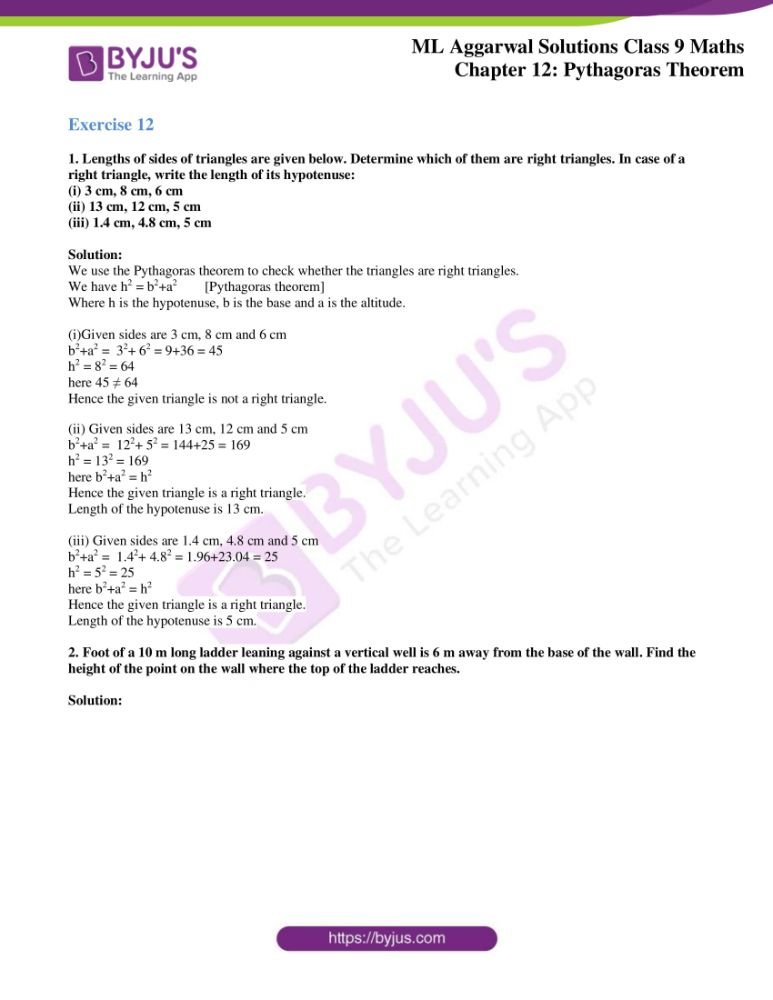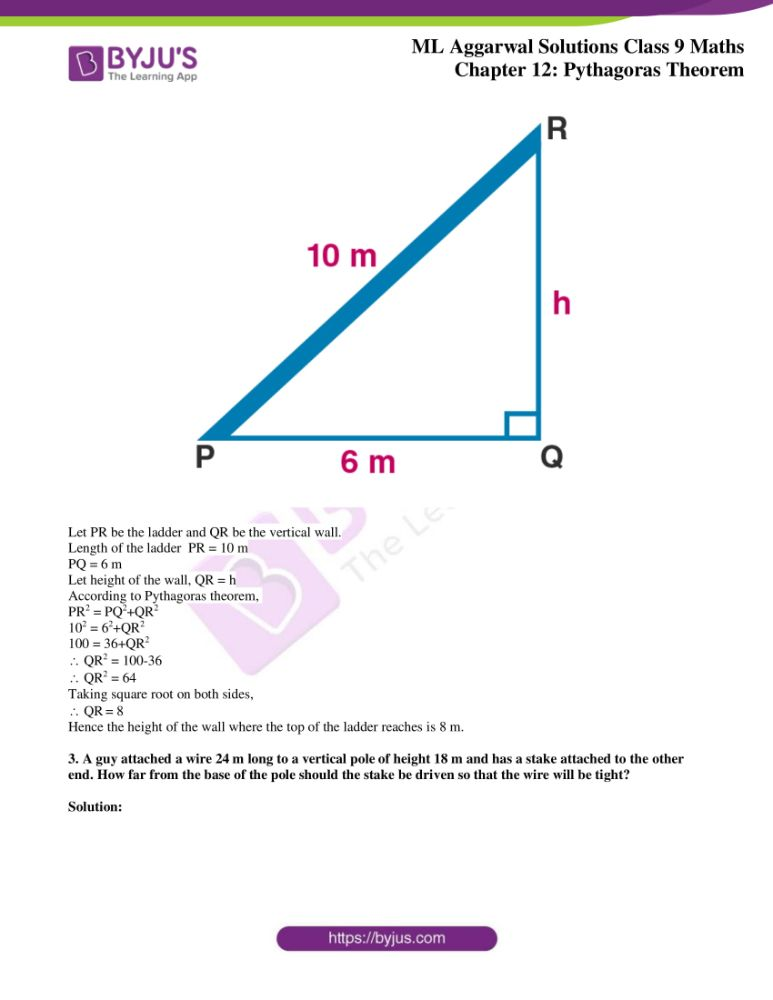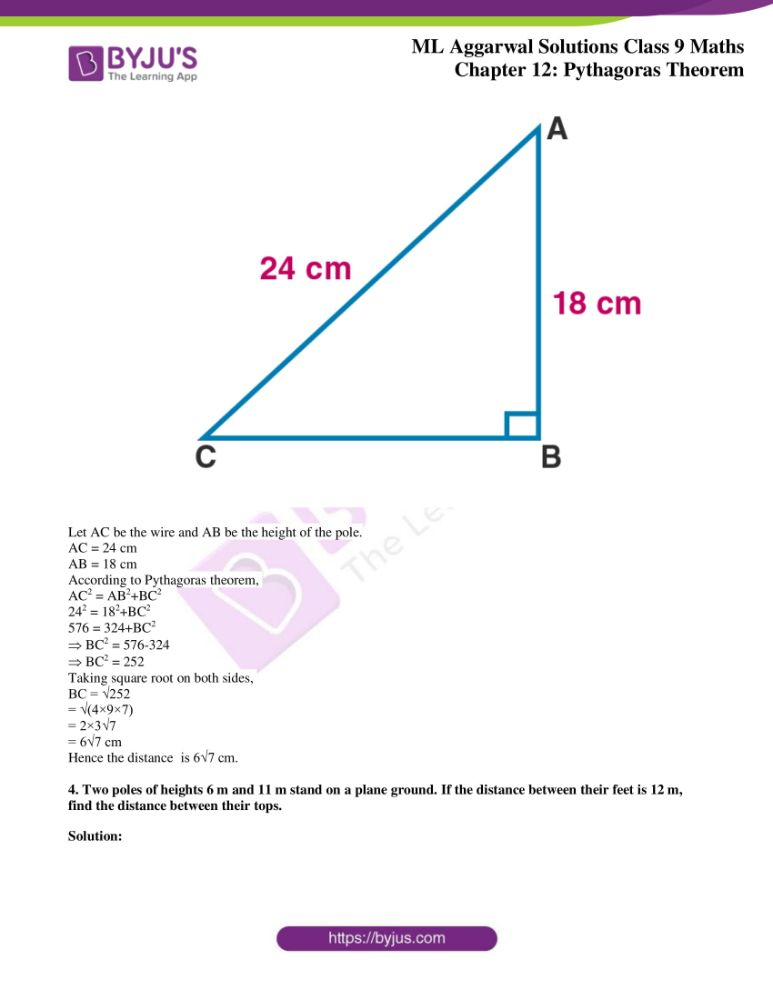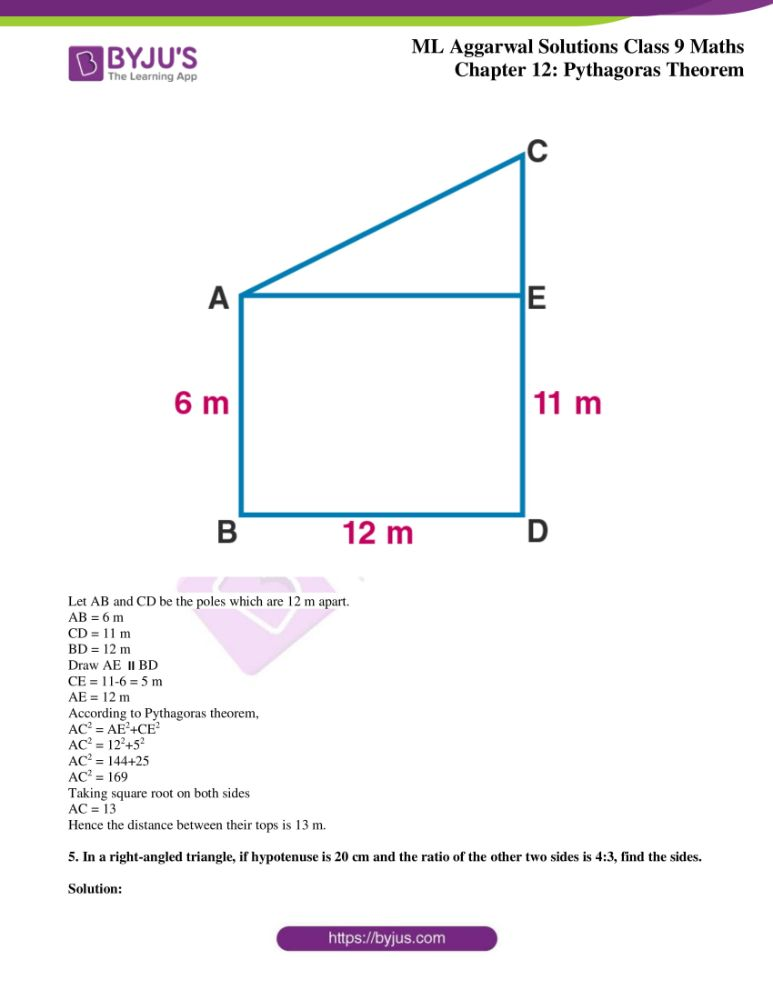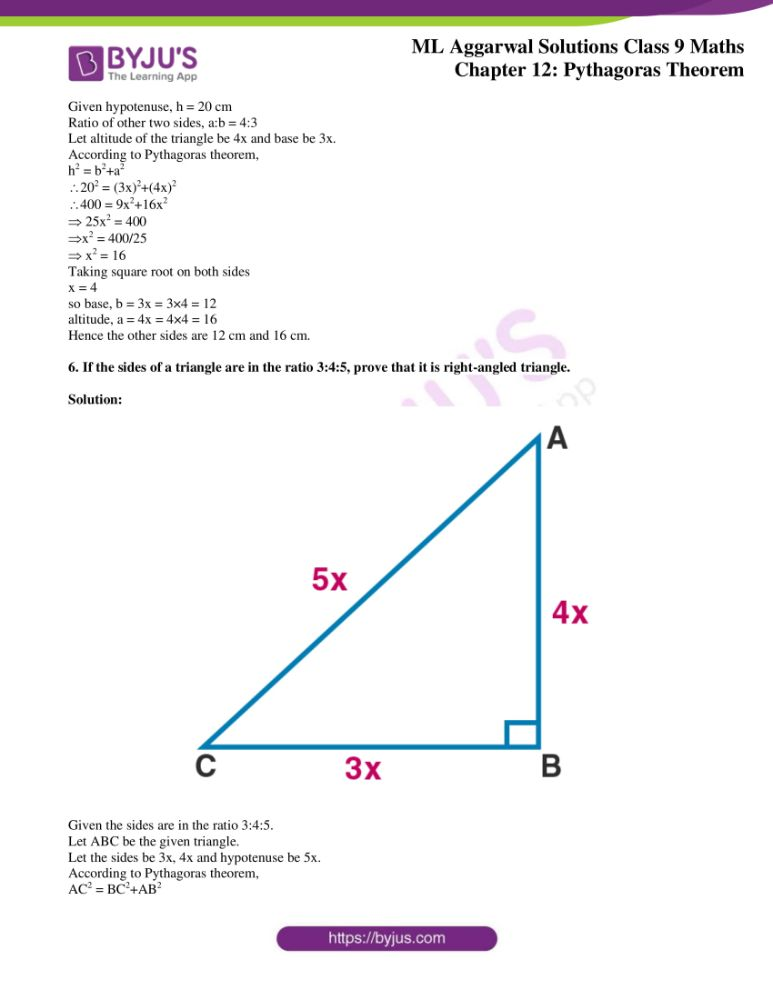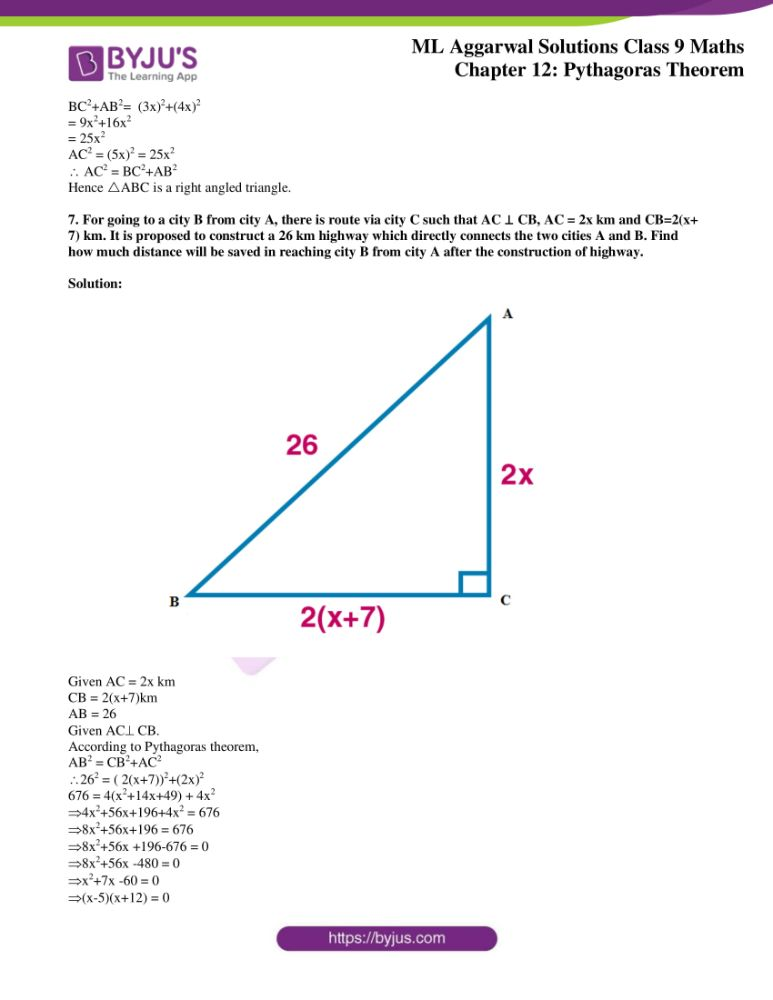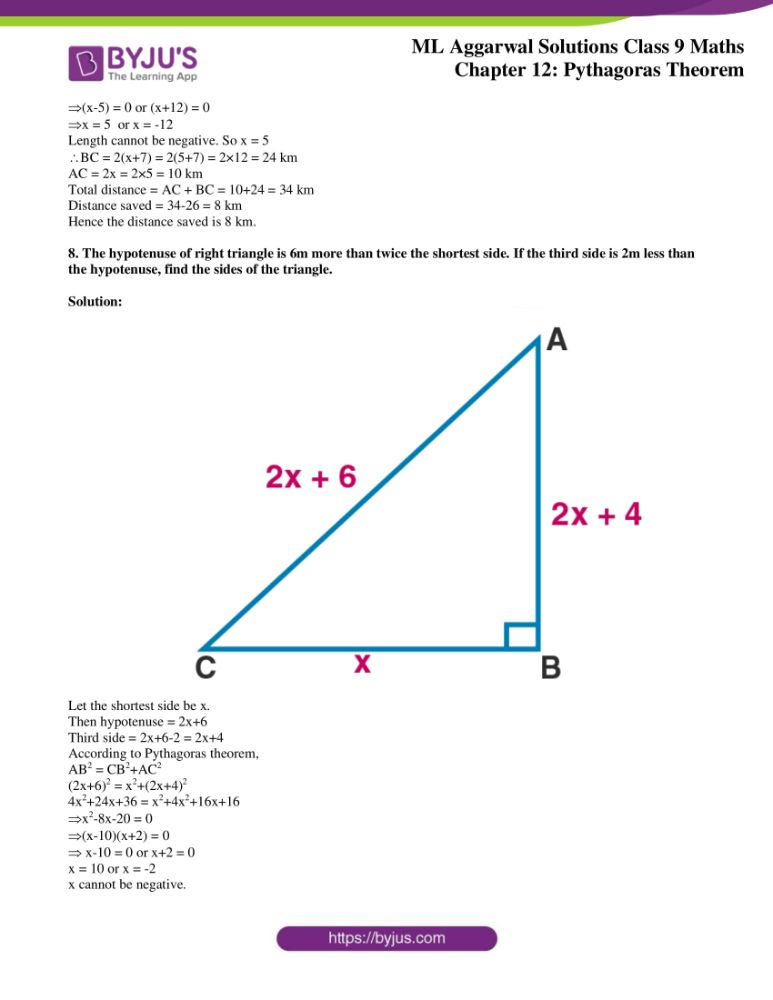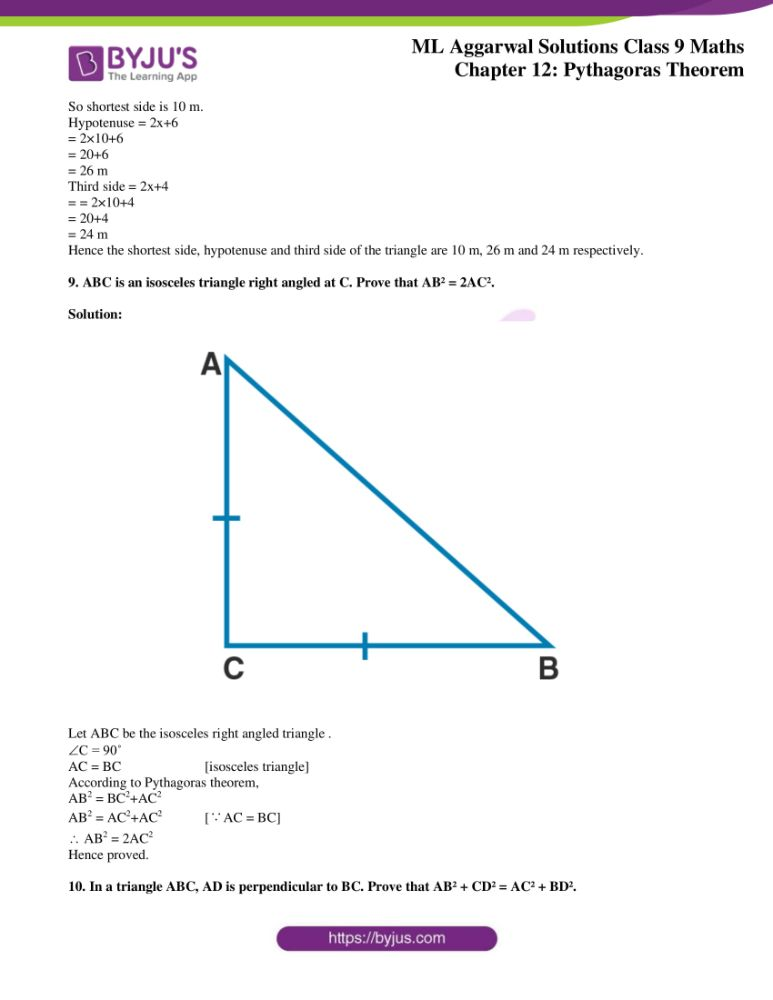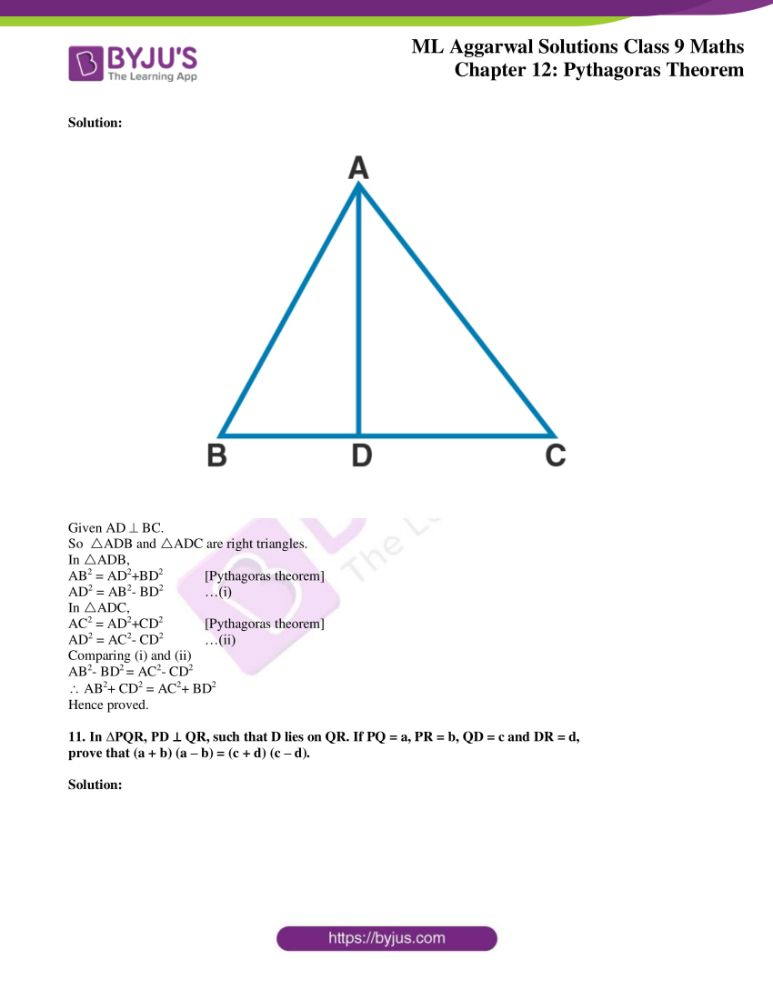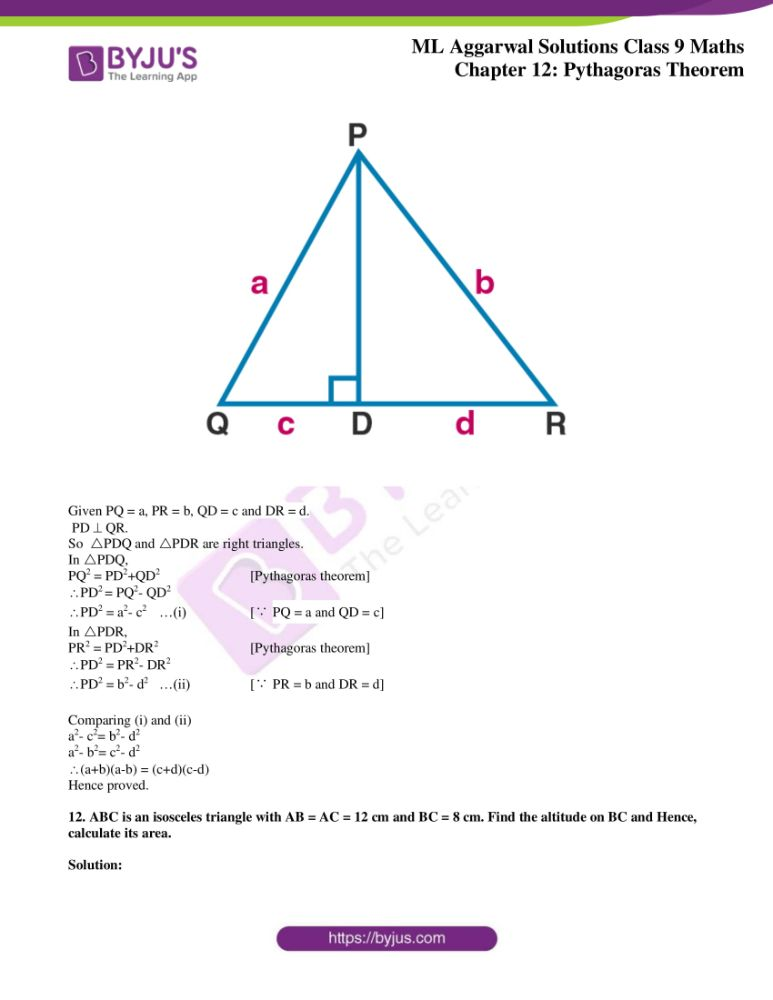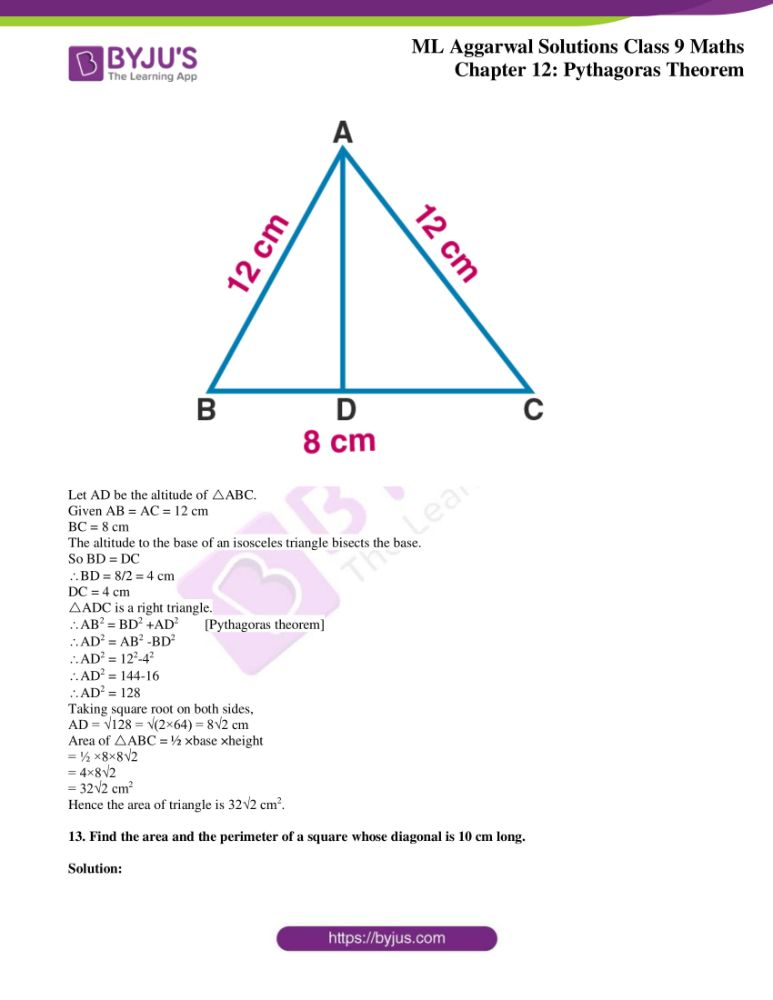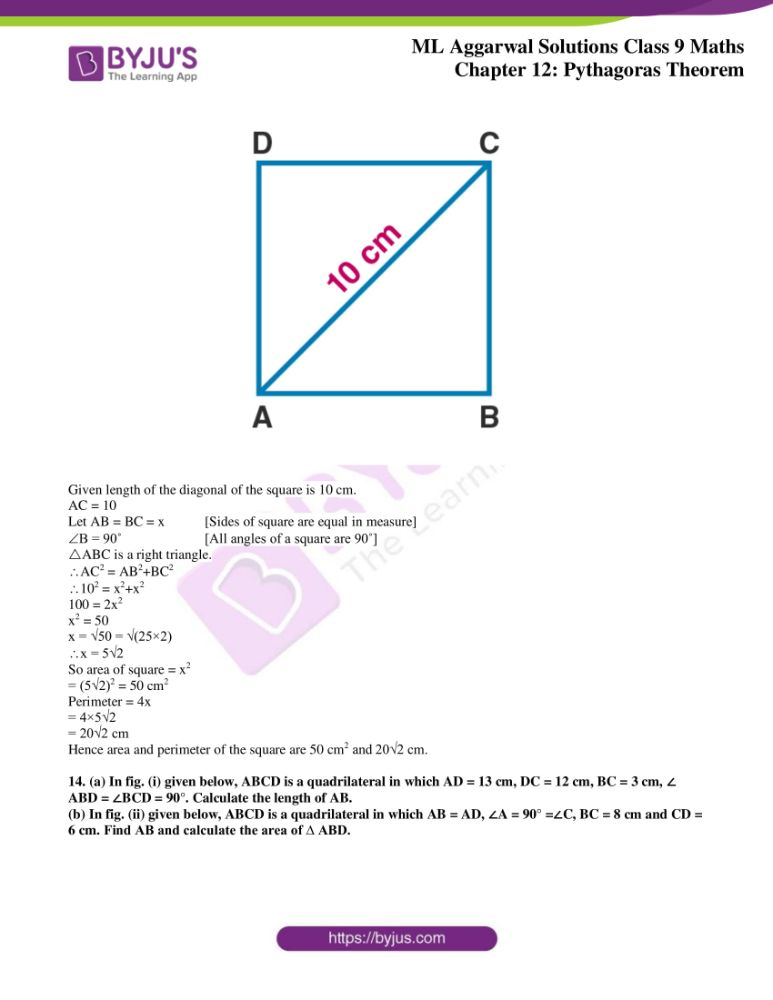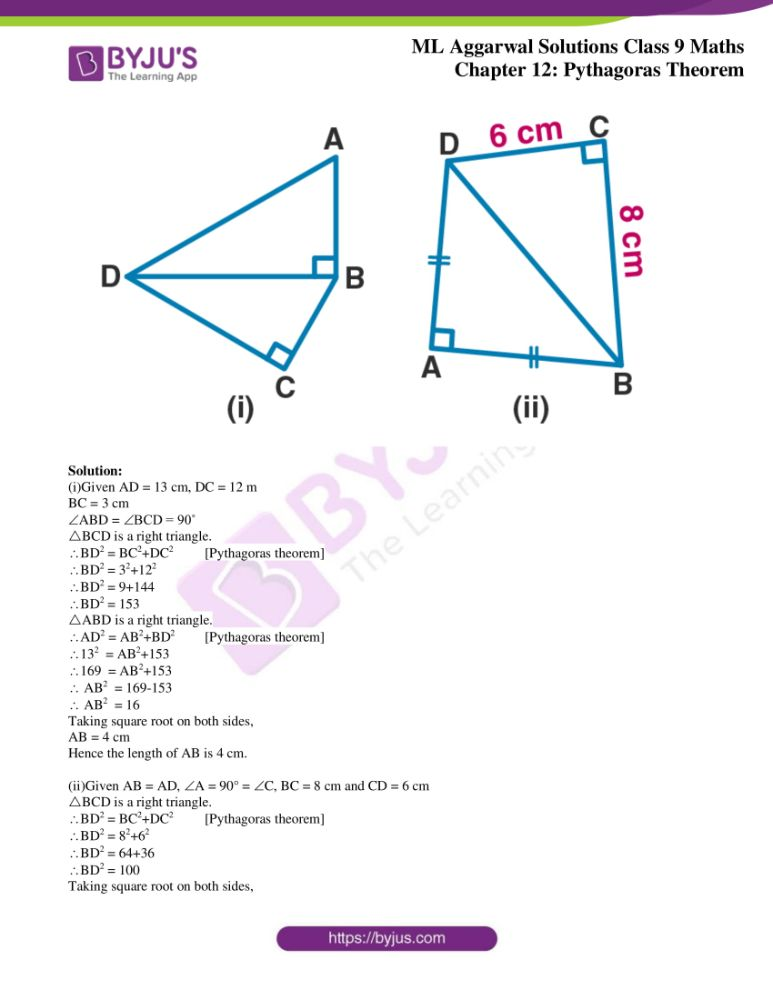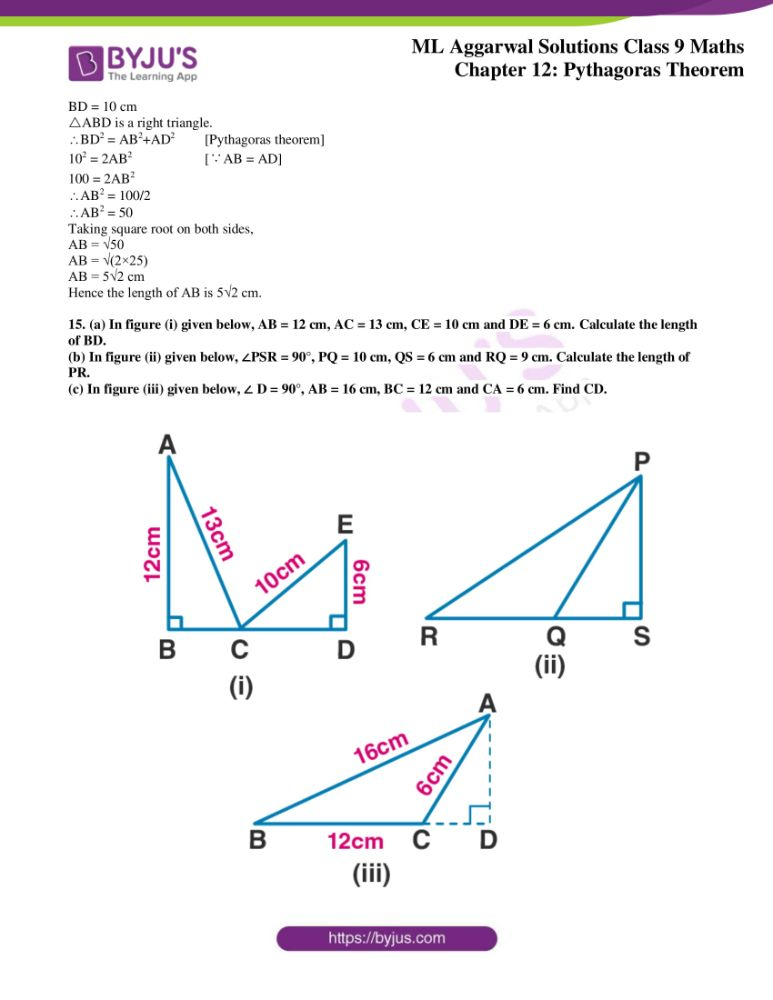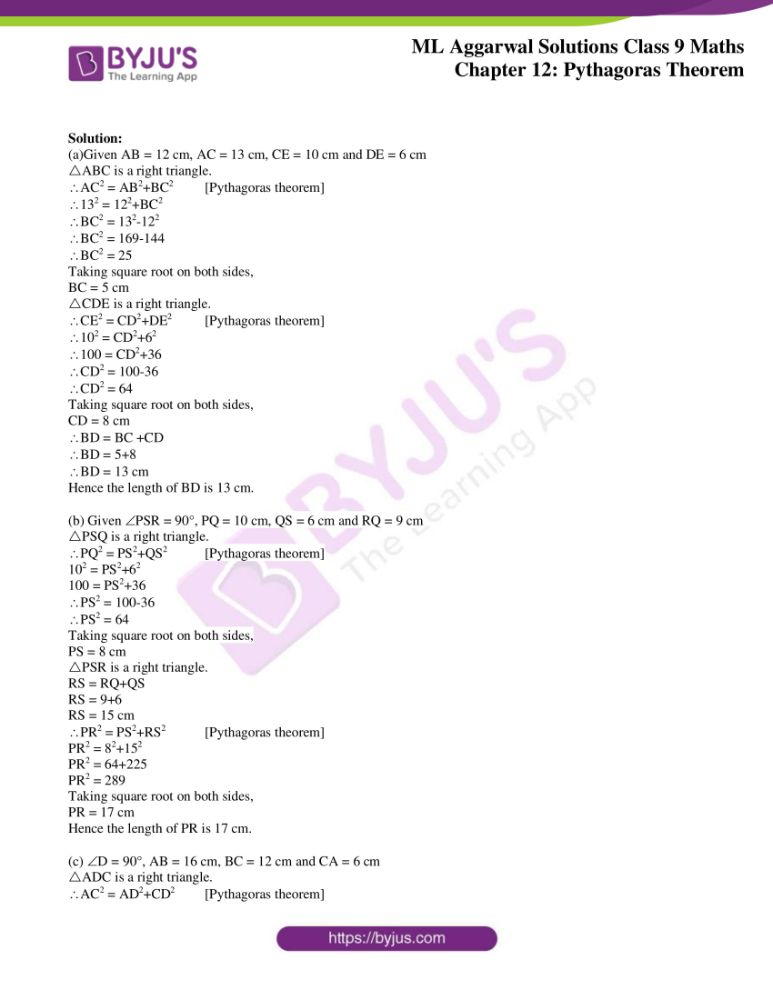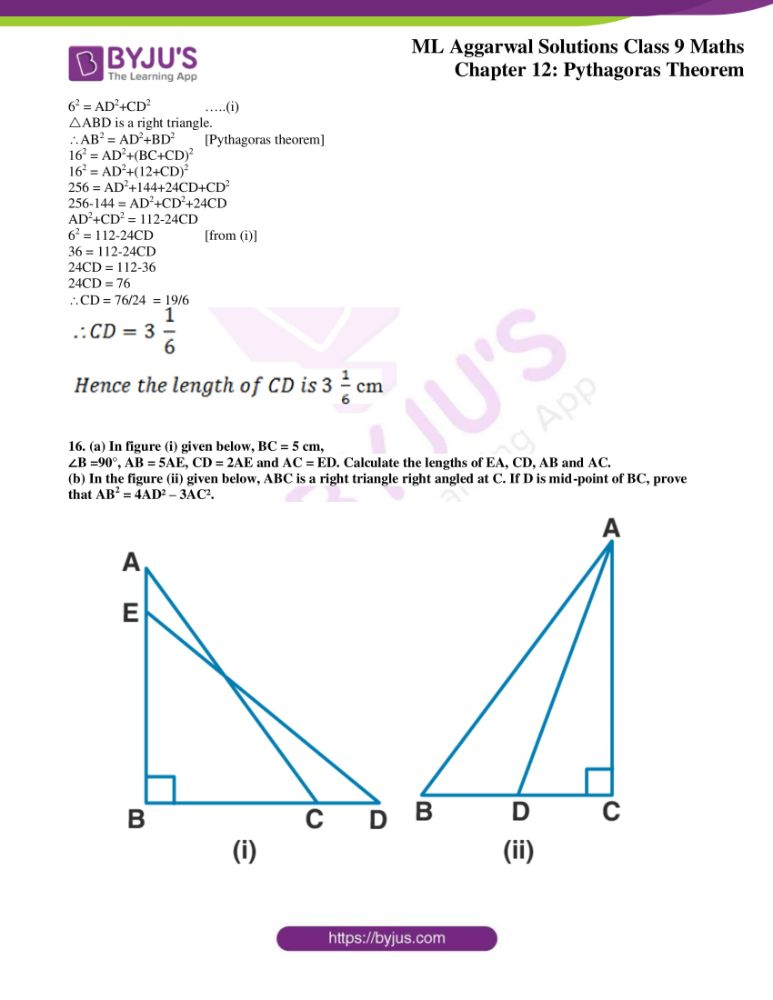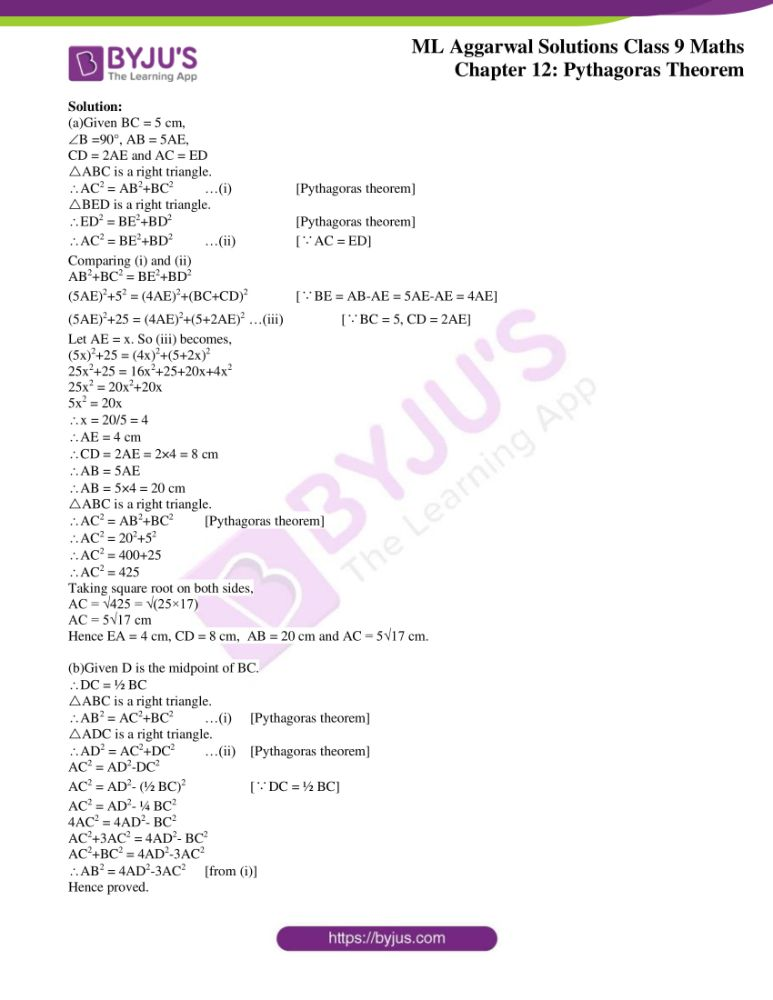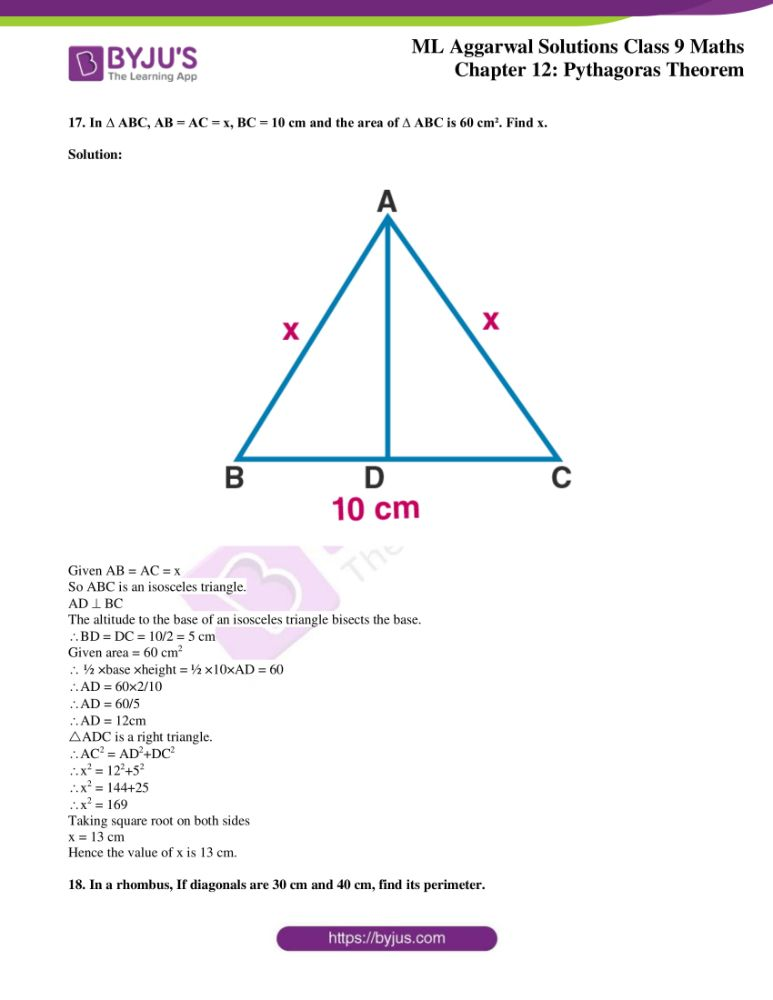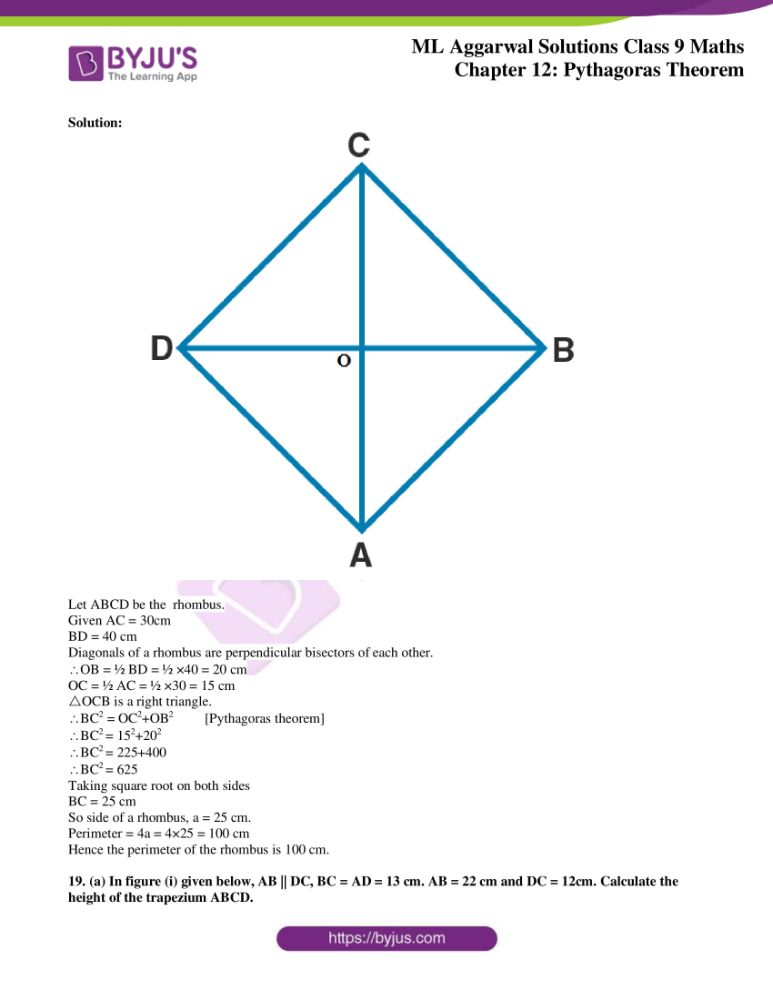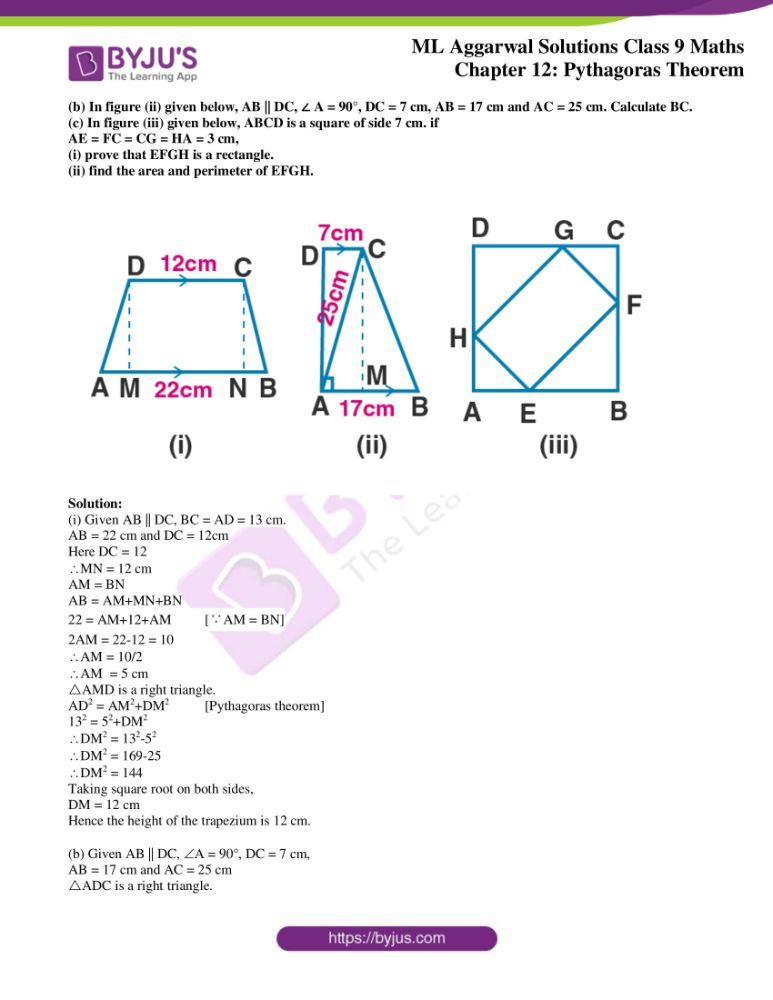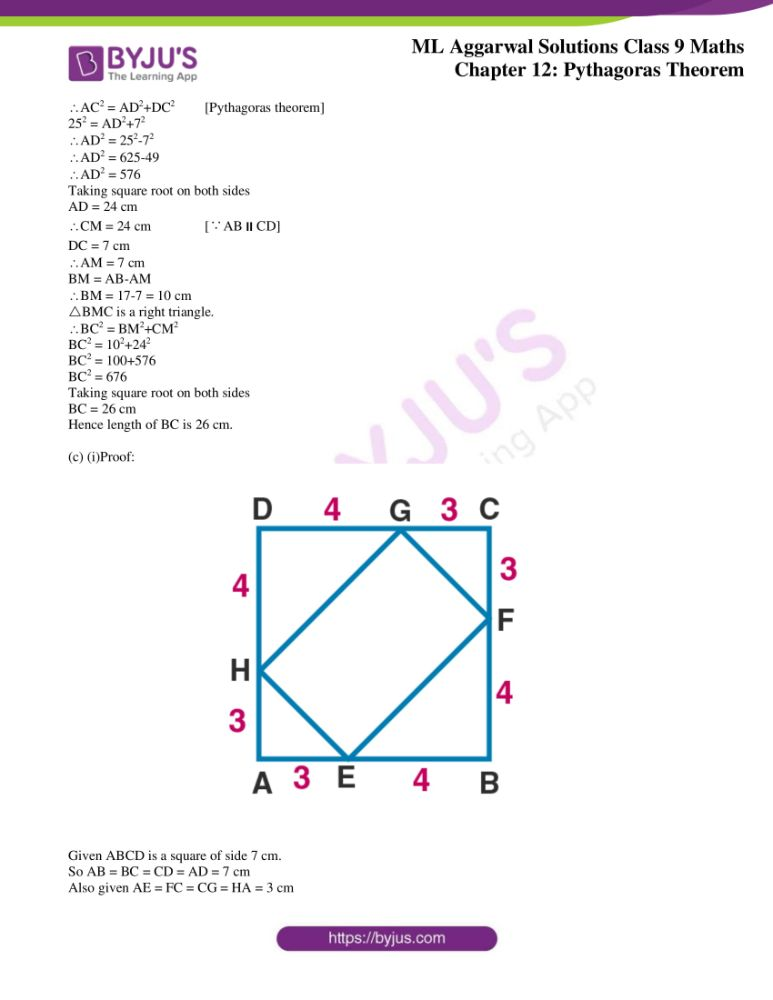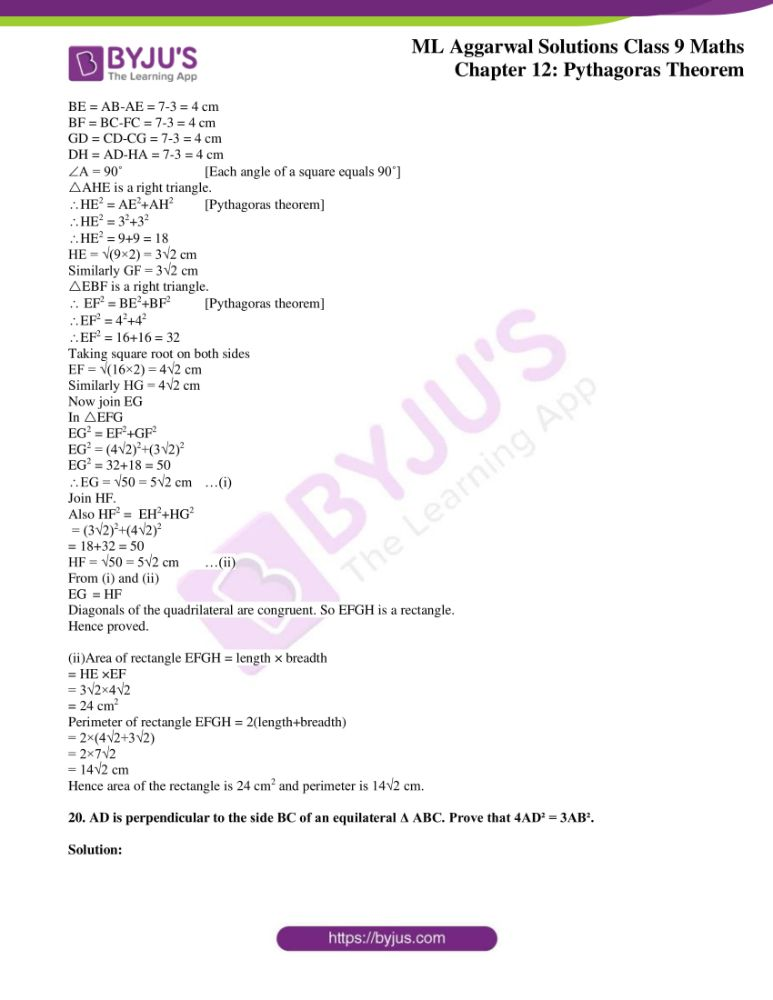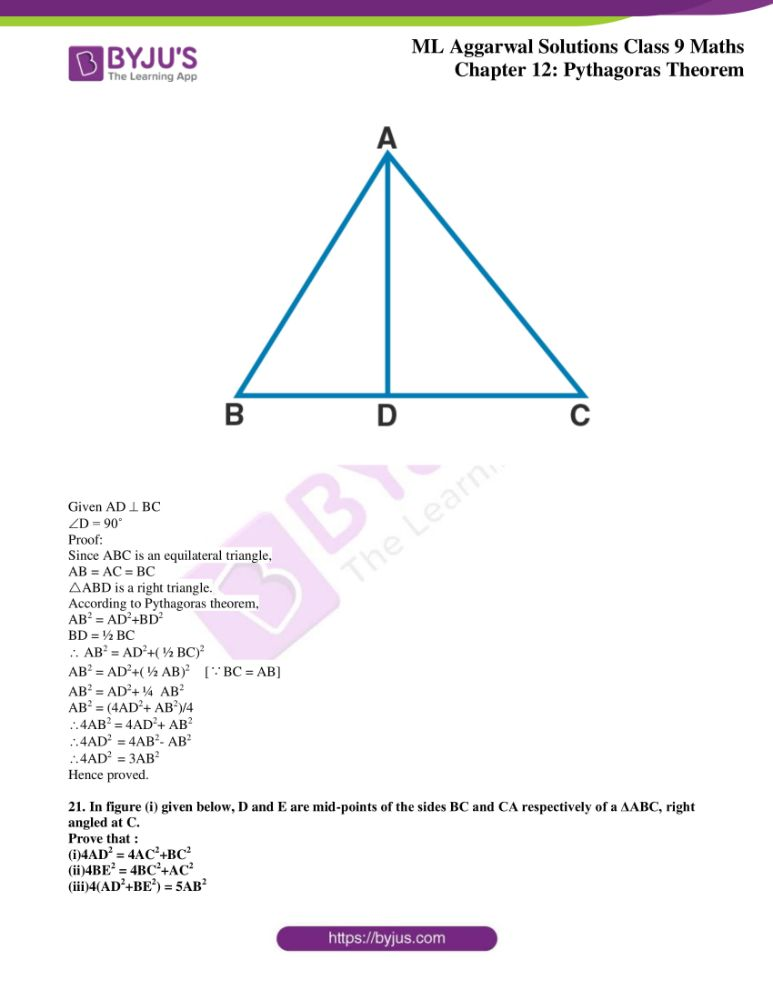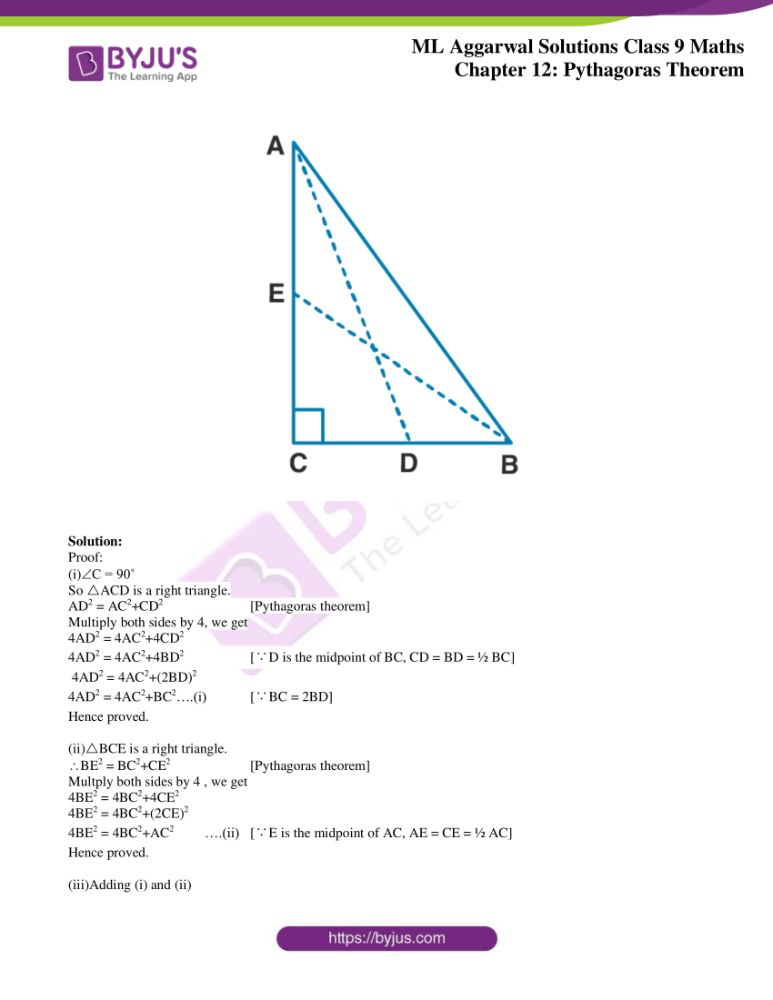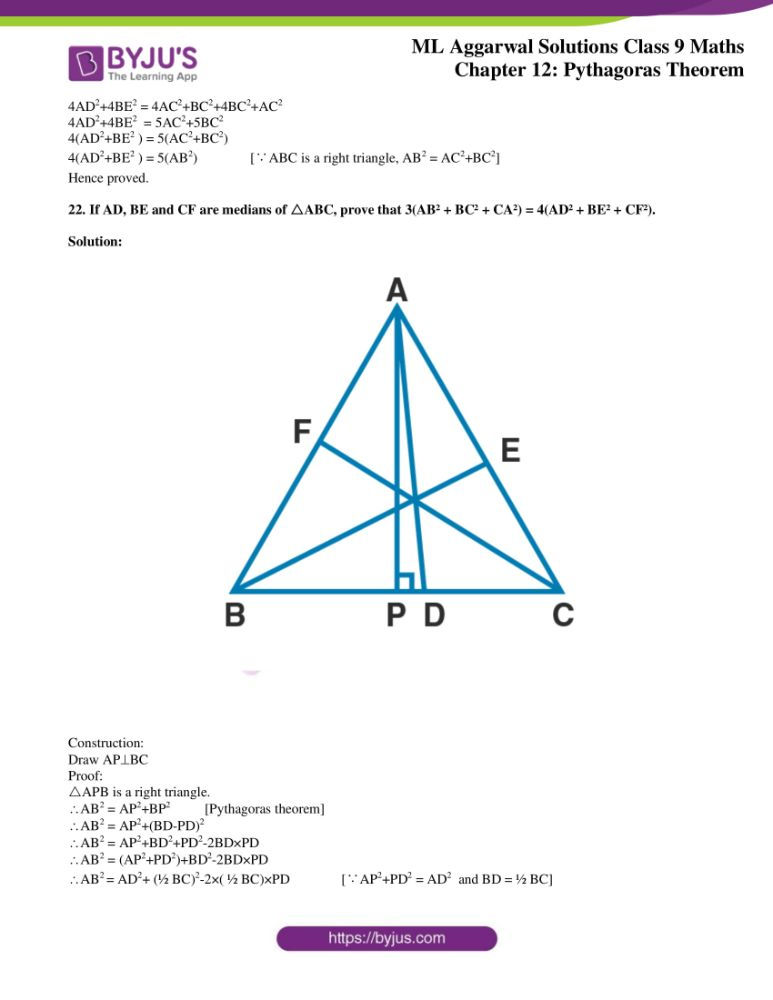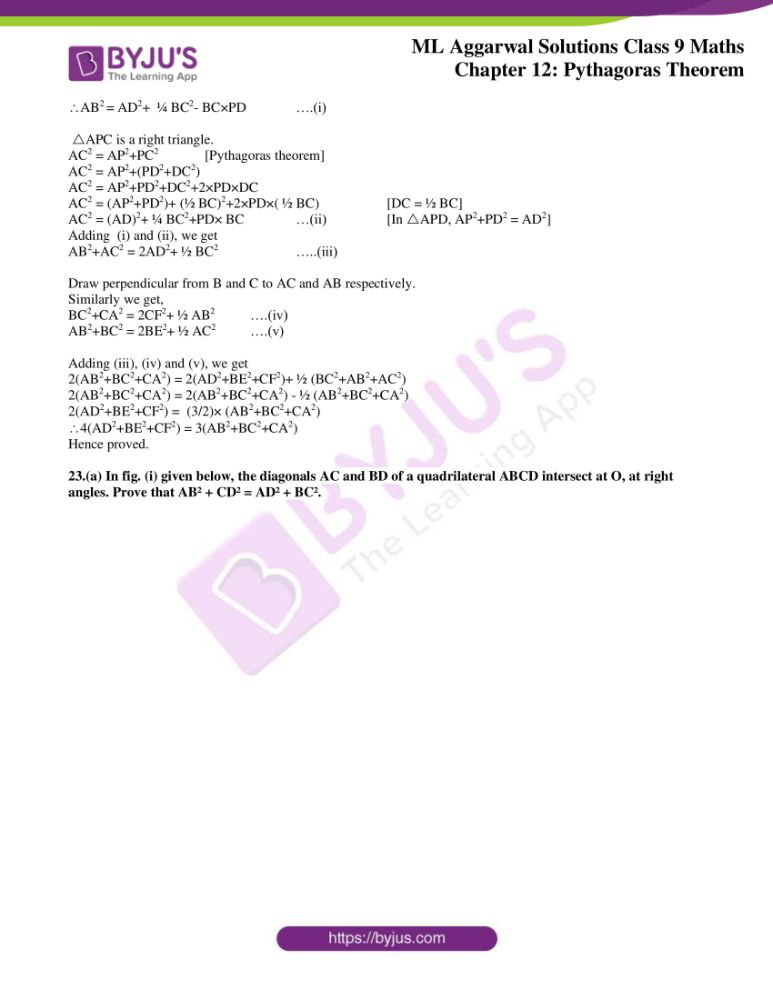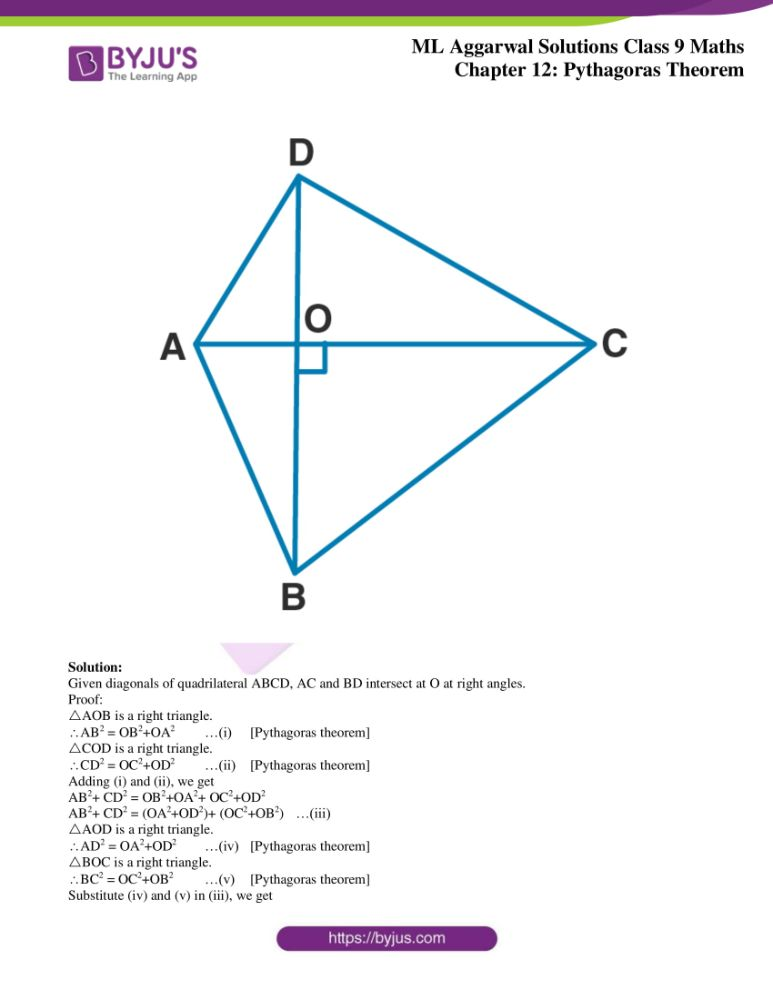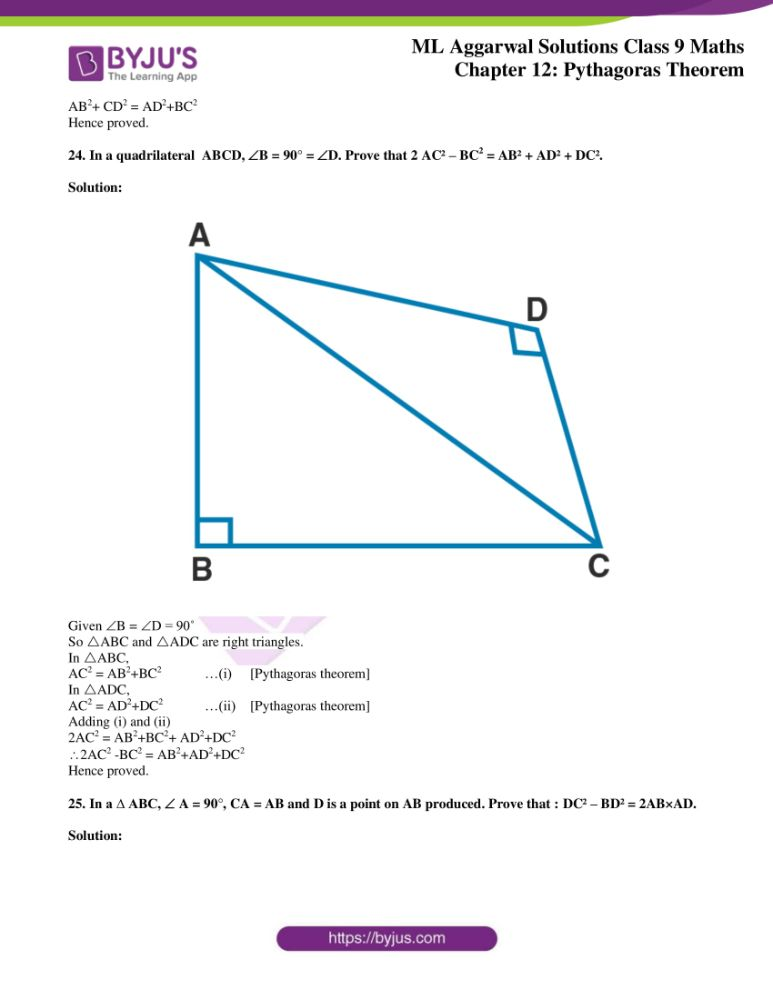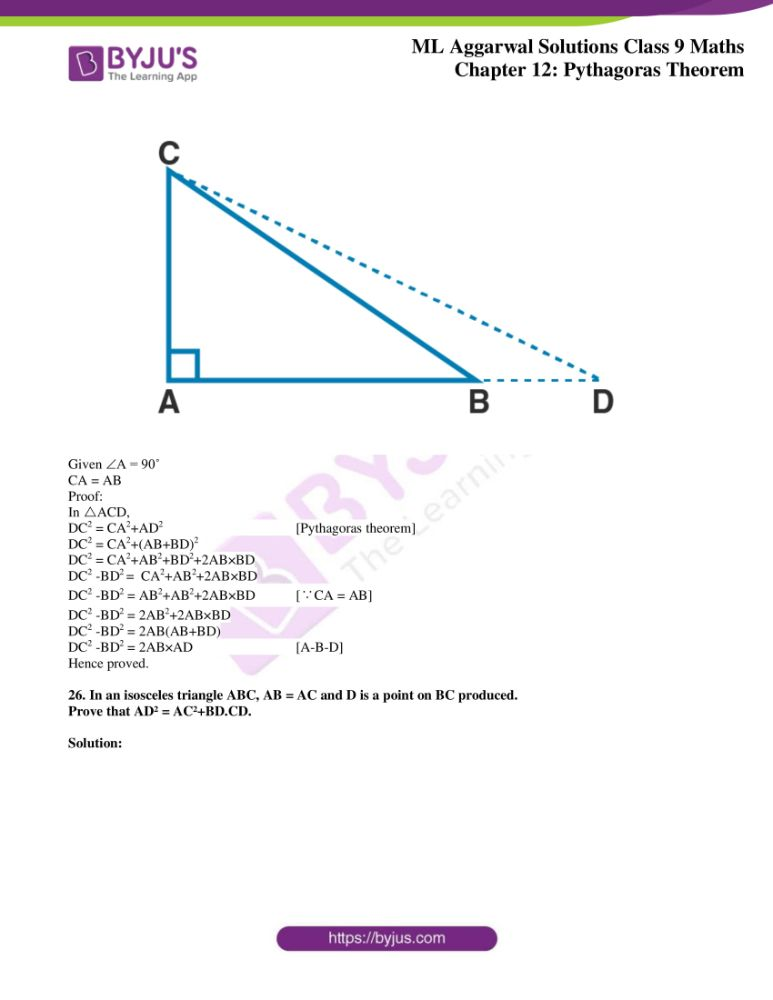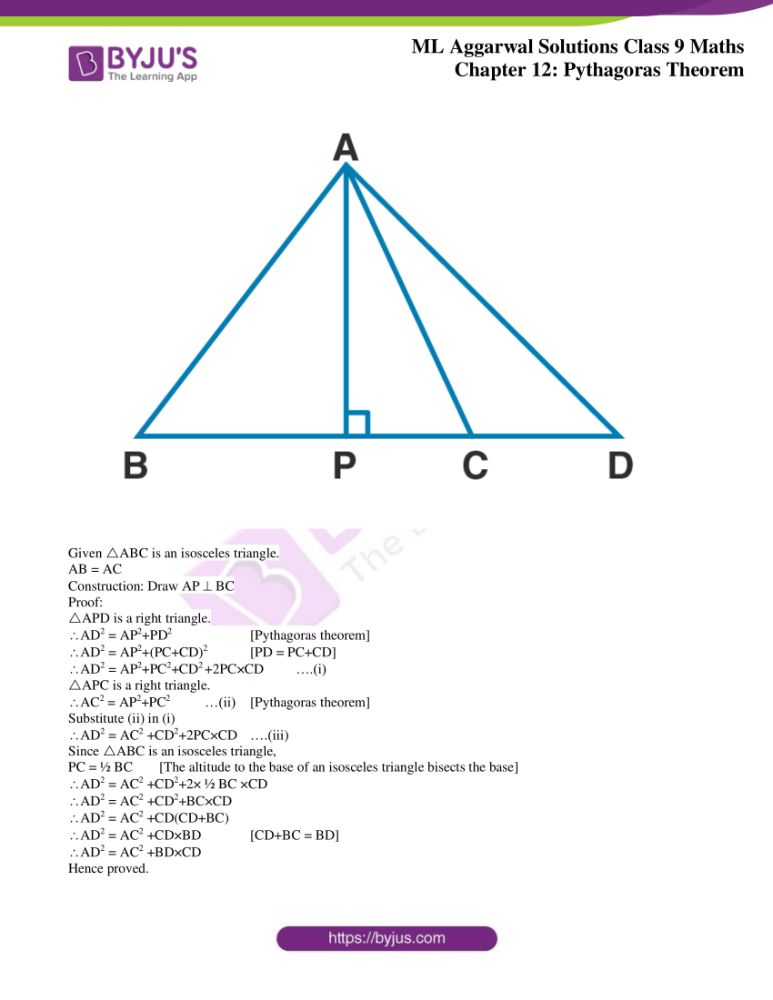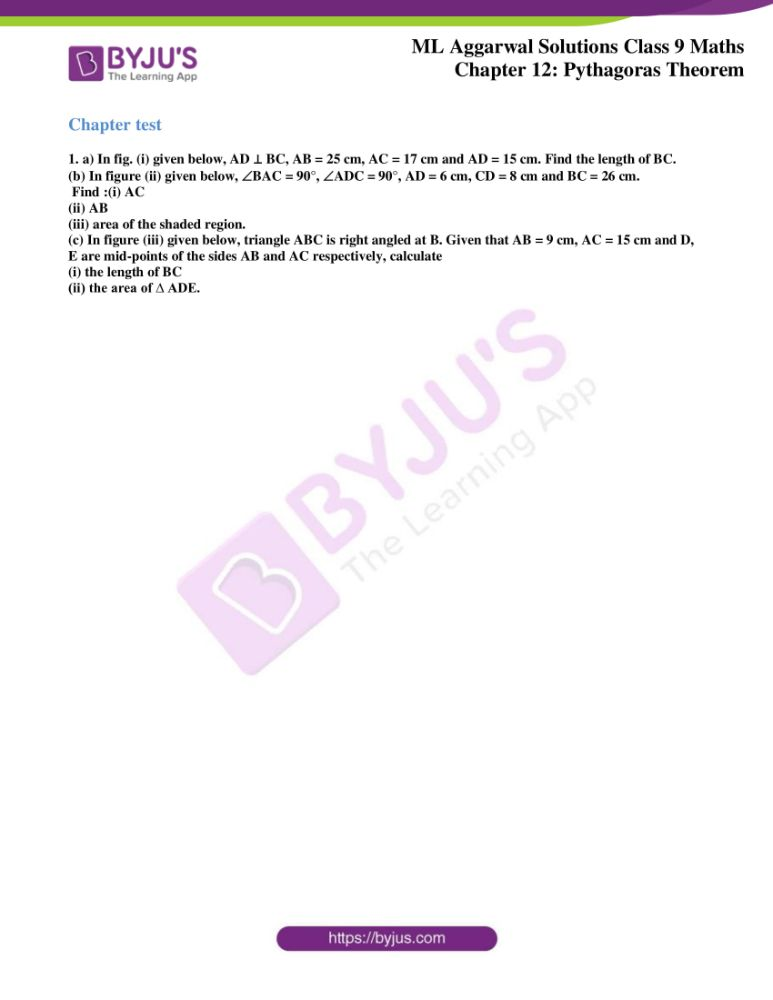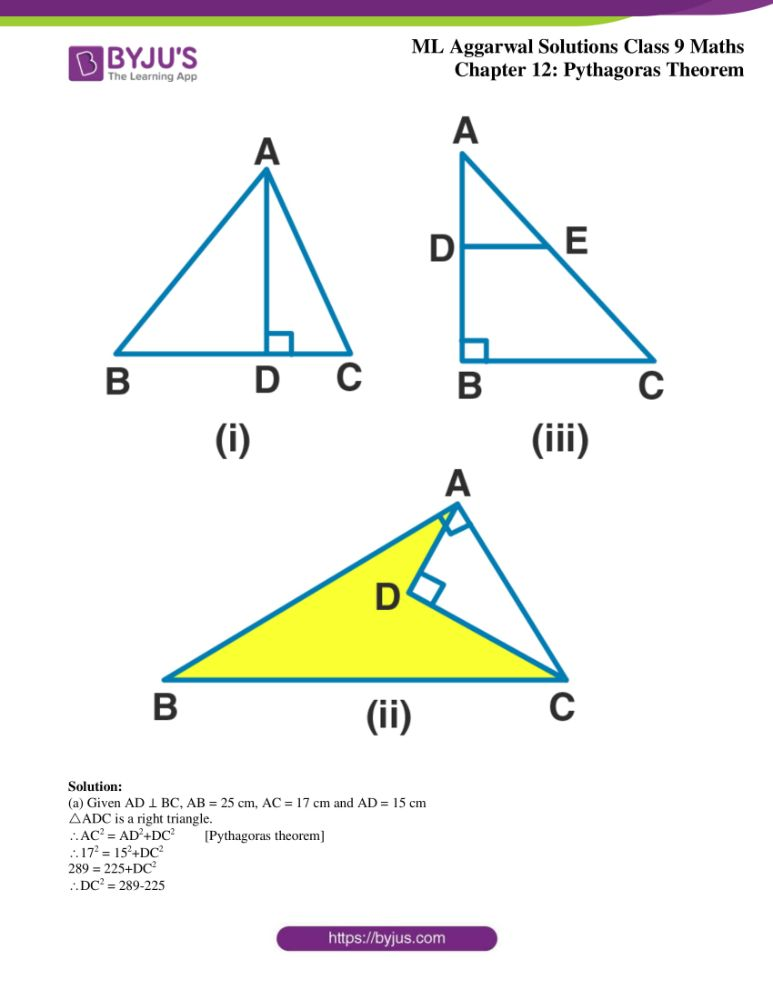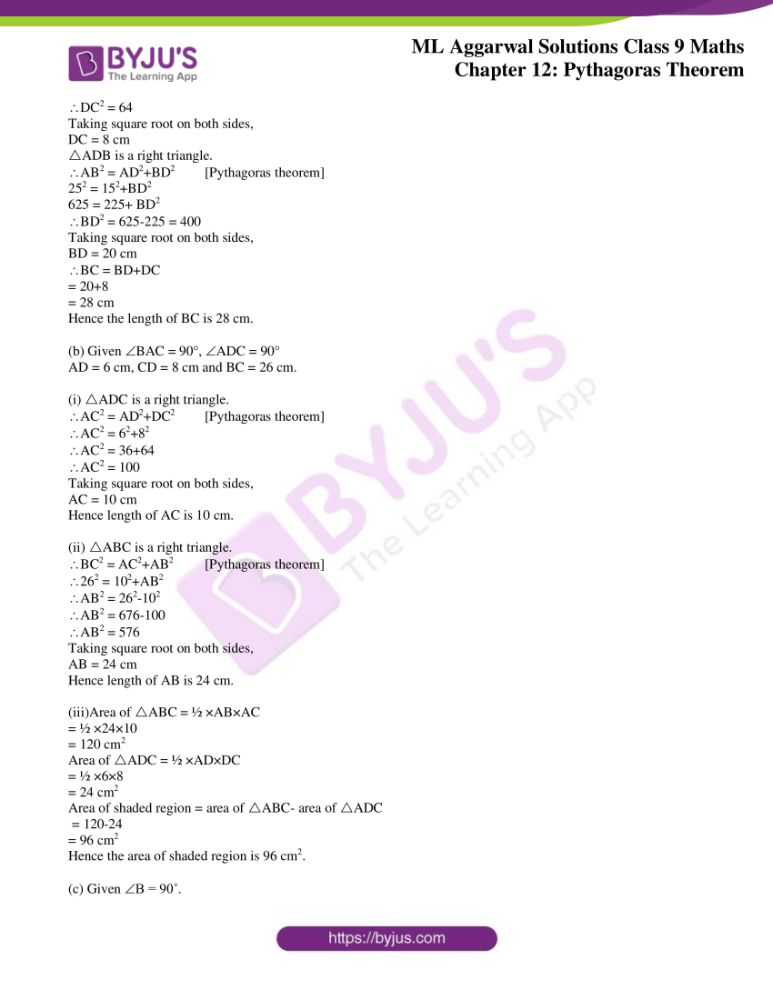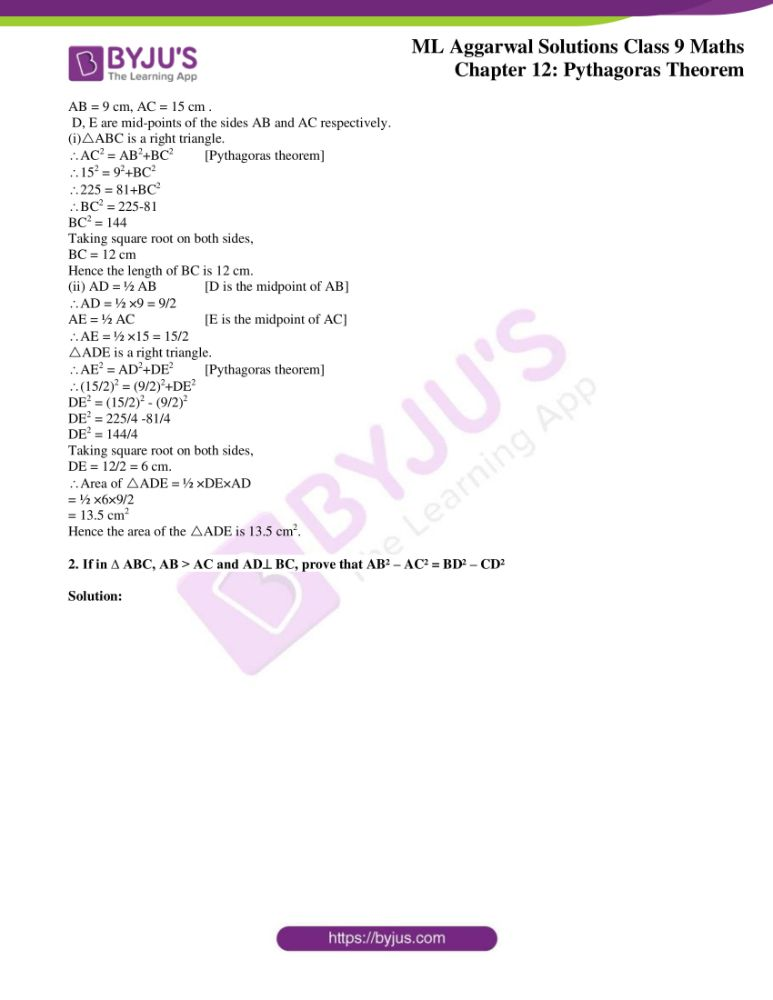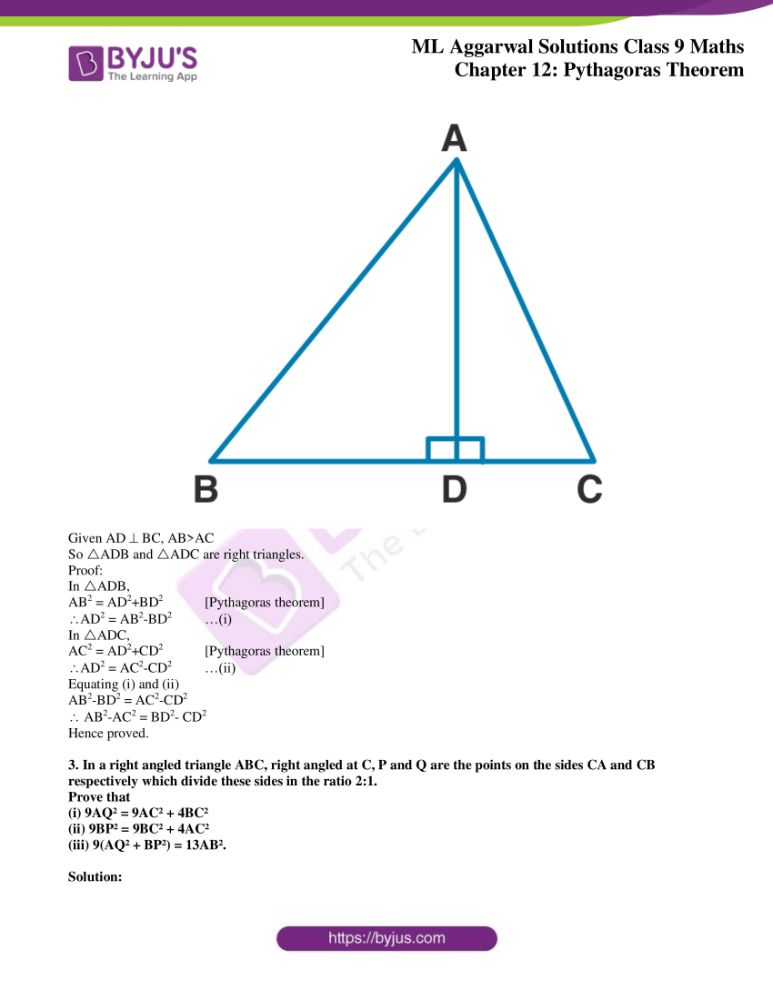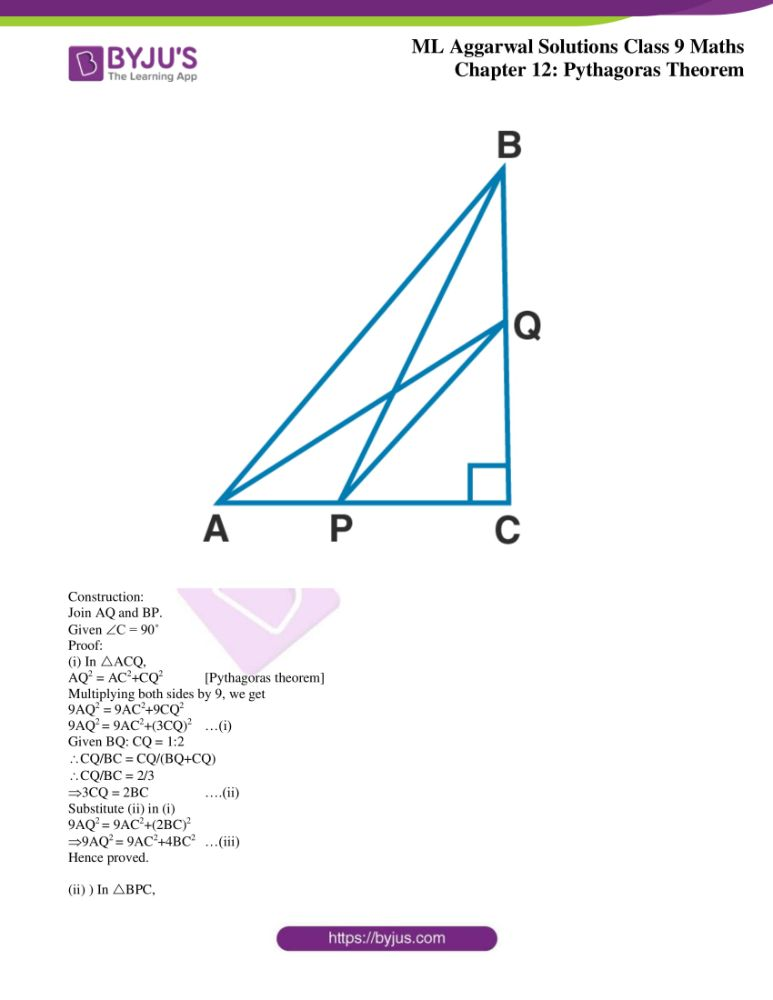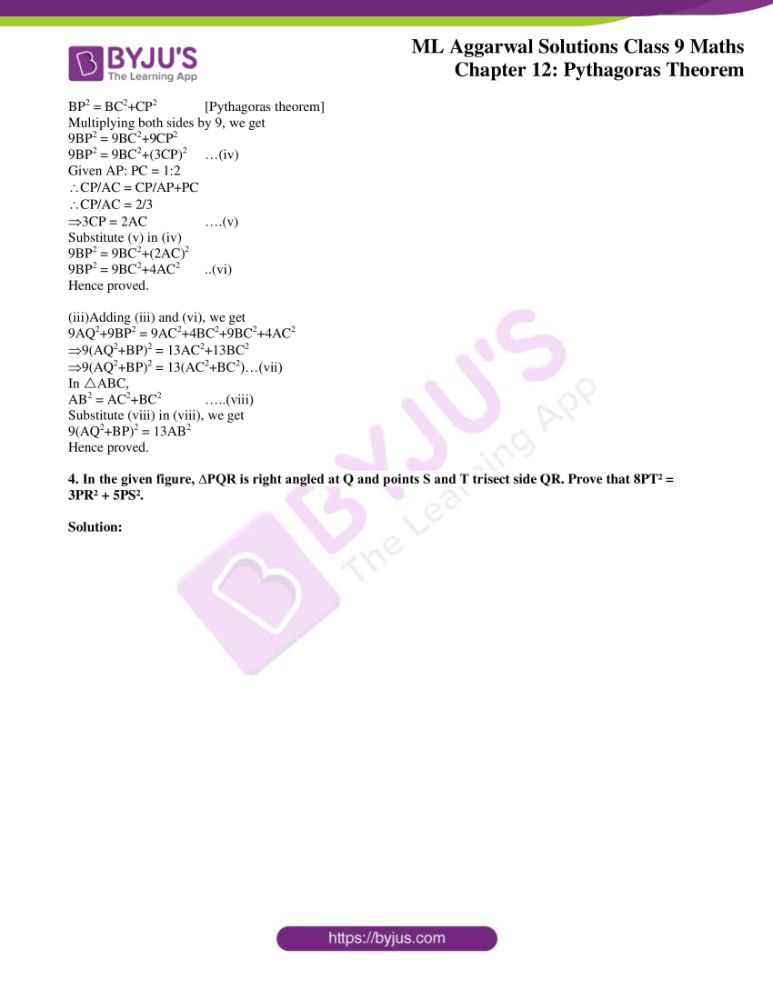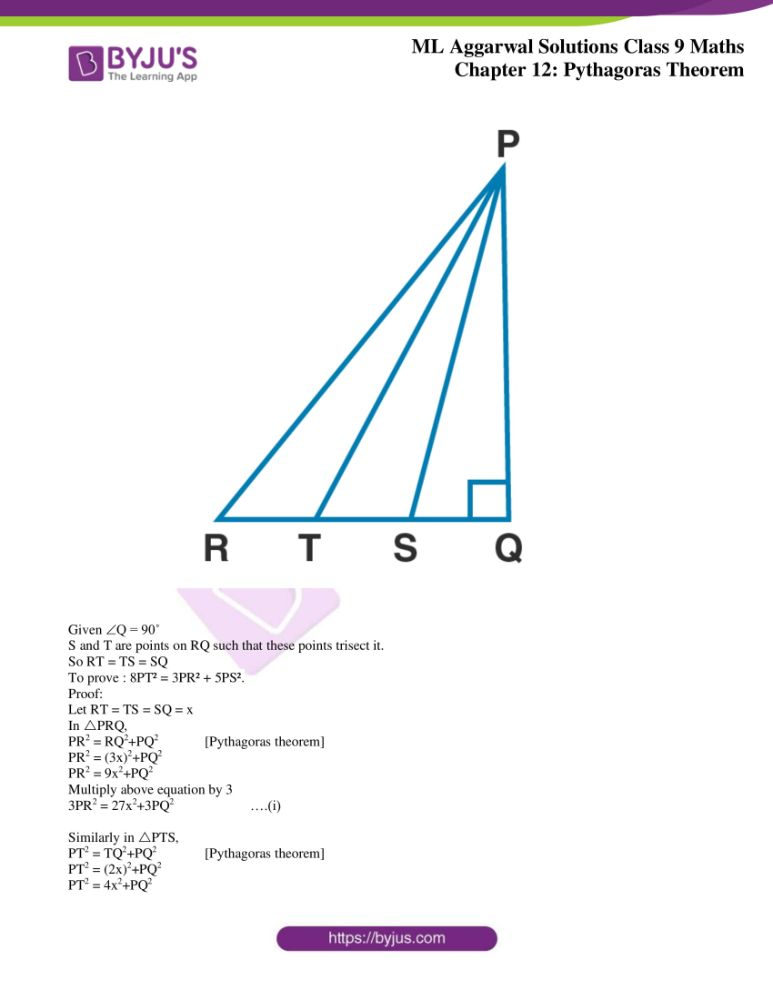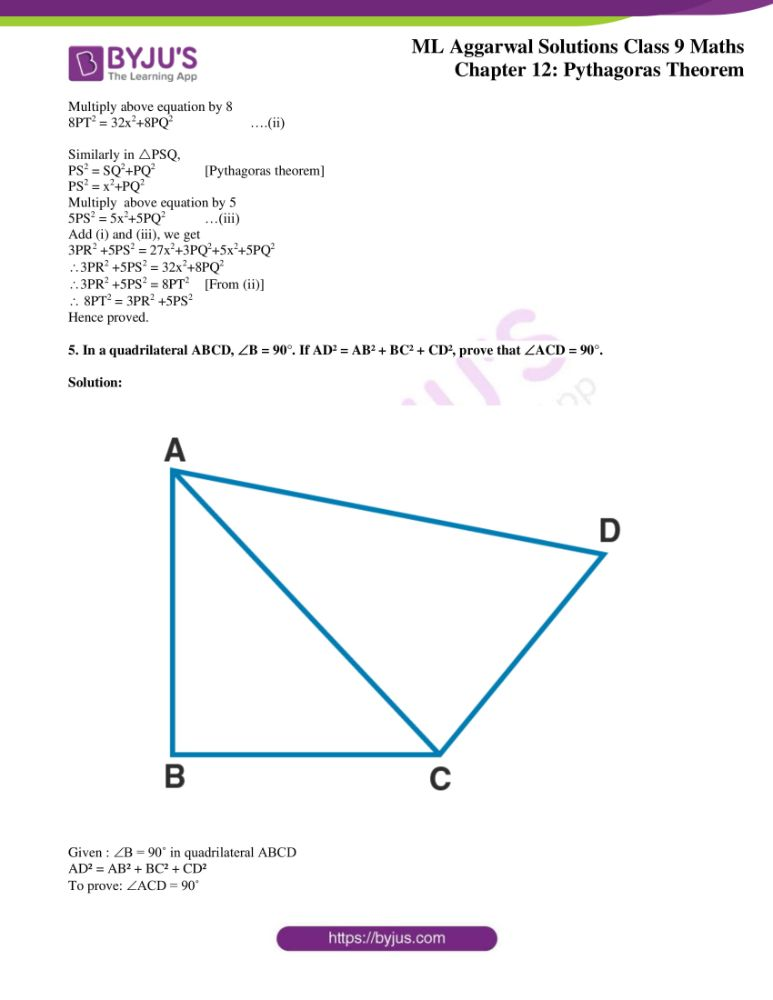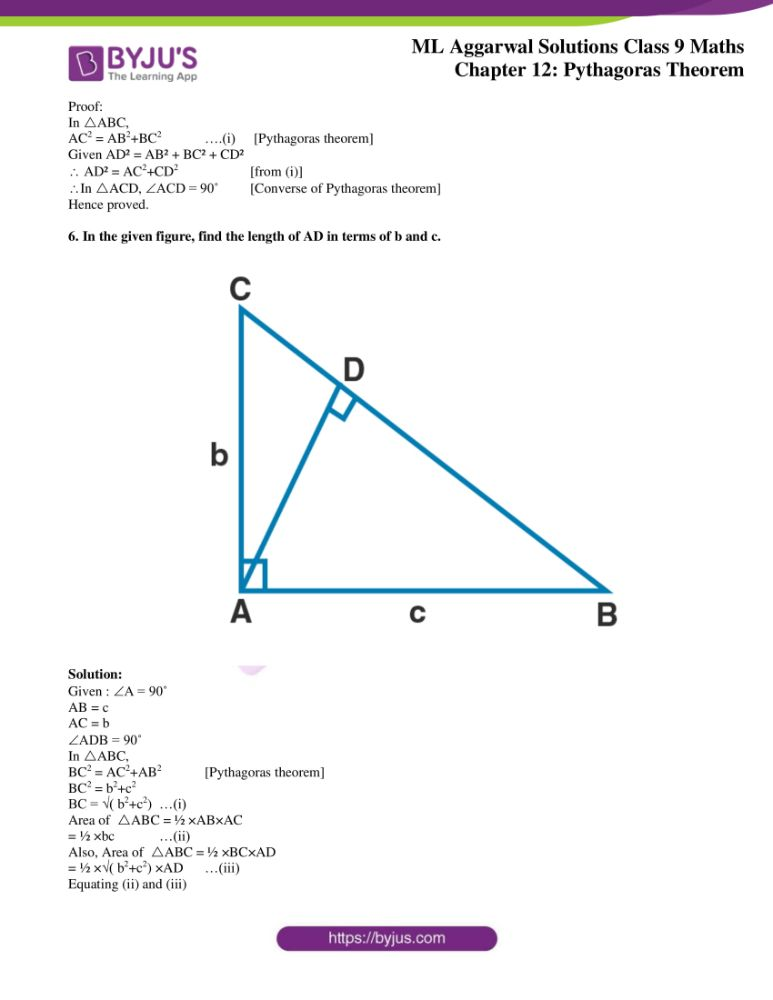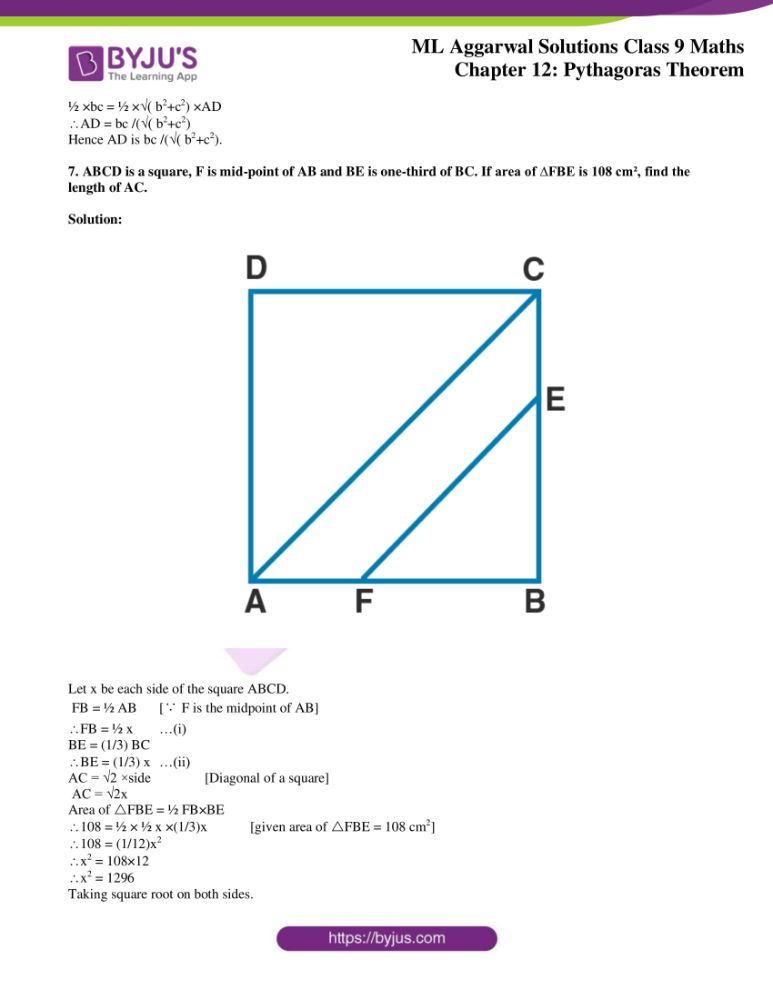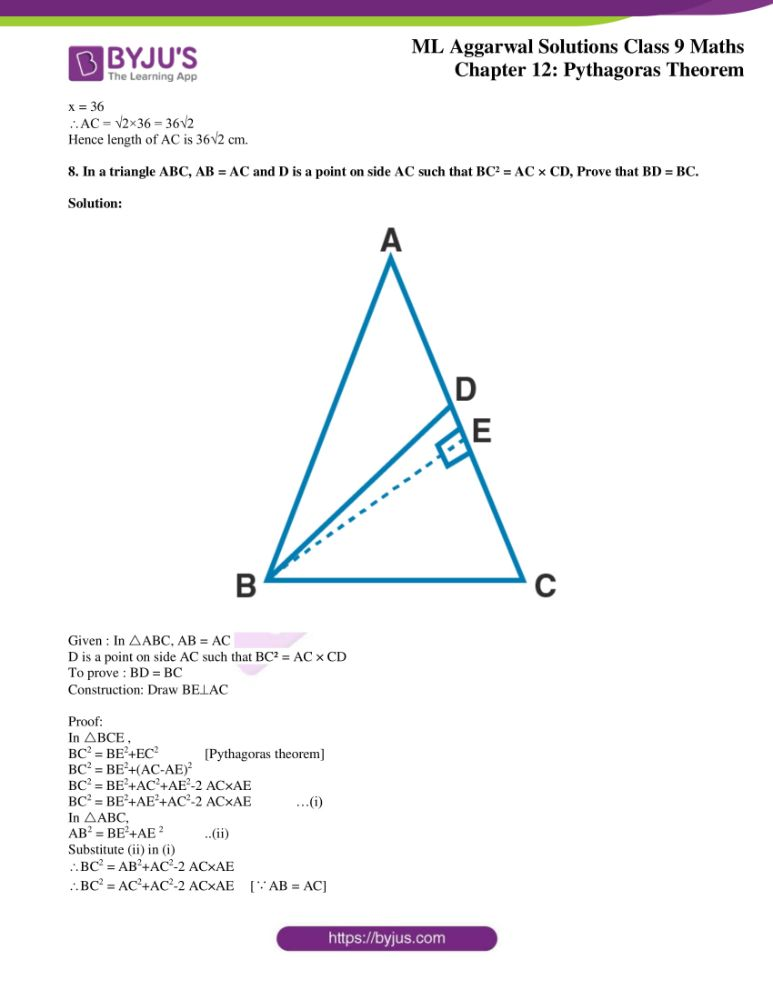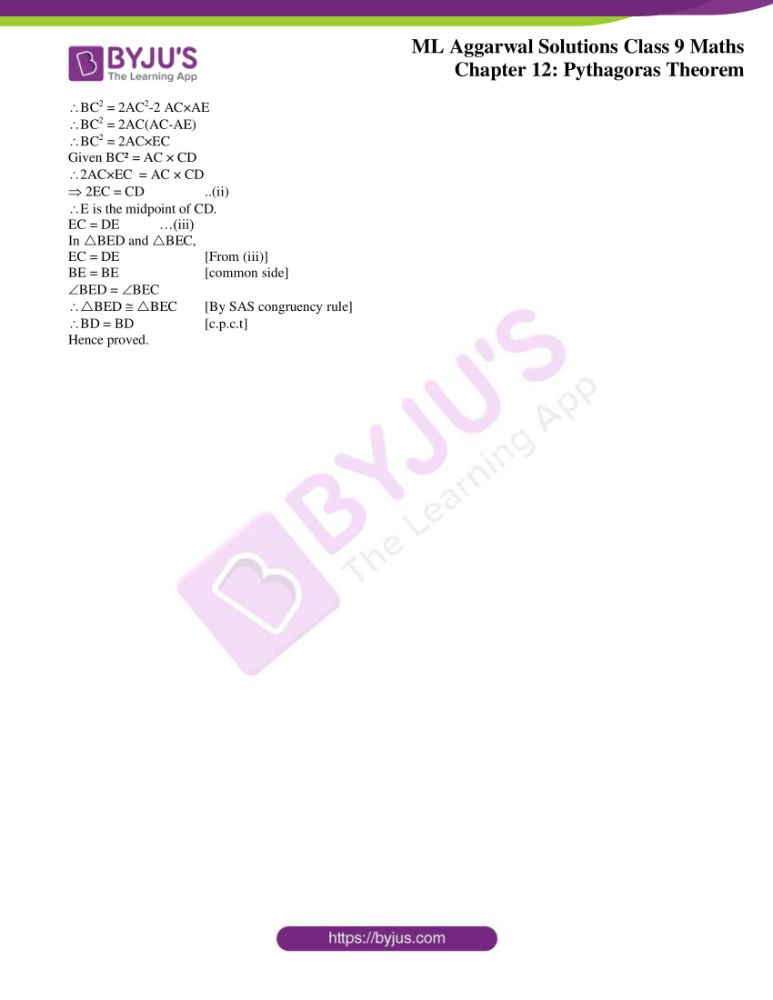### Access answers to ML Aggarwal Solutions for Class 9 Maths Chapter 12 – Pythagoras Theorem

Exercise 12

1. Lengths of sides of triangles are given below. Determine which of them are right triangles. In case of a right triangle, write the length of its hypotenuse:

(i) 3 cm, 8 cm, 6 cm

(ii) 13 cm, 12 cm, 5 cm

(iii) 1.4 cm, 4.8 cm, 5 cm

Solution:

We use the Pythagoras theorem to check whether the triangles are right triangles.

We have h2 = b2+a2 [Pythagoras theorem]

Where h is the hypotenuse, b is the base and a is the altitude.

(i)Given sides are 3 cm, 8 cm and 6 cm

b2+a2 = 32+ 62 = 9+36 = 45

h2 = 82 = 64

here 45 ≠ 64

Hence the given triangle is not a right triangle.

(ii) Given sides are 13 cm, 12 cm and 5 cm

b2+a2 = 122+ 52 = 144+25 = 169

h2 = 132 = 169

here b2+a2 = h2

Hence the given triangle is a right triangle.

Length of the hypotenuse is 13 cm.

(iii) Given sides are 1.4 cm, 4.8 cm and 5 cm

b2+a2 = 1.42+ 4.82 = 1.96+23.04 = 25

h2 = 52 = 25

here b2+a2 = h2

Hence the given triangle is a right triangle.

Length of the hypotenuse is 5 cm.

2. Foot of a 10 m long ladder leaning against a vertical well is 6 m away from the base of the wall. Find the height of the point on the wall where the top of the ladder reaches.

Solution: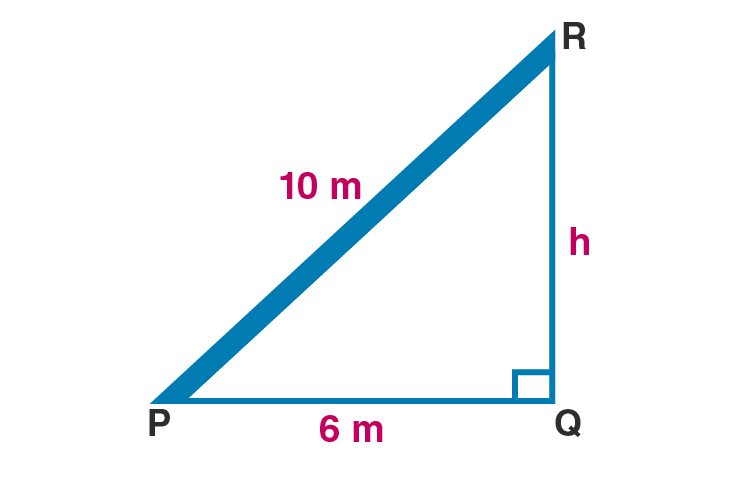Let PR be the ladder and QR be the vertical wall.

Length of the ladder PR = 10 m

PQ = 6 m

Let height of the wall, QR = h

According to Pythagoras theorem,

PR2 = PQ2+QR2

102 = 62+QR2

100 = 36+QR2

QR2 = 100-36

QR2 = 64

Taking square root on both sides,

QR = 8

Hence the height of the wall where the top of the ladder reaches is 8 m.

3. A guy attached a wire 24 m long to a vertical pole of height 18 m and has a stake attached to the other end. How far from the base of the pole should the stake be driven so that the wire will be tight?

Solution: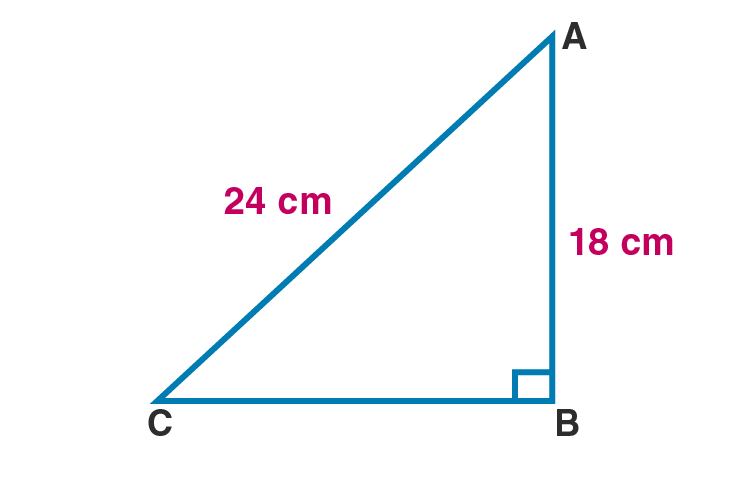Let AC be the wire and AB be the height of the pole.

AC = 24 cm

AB = 18 cm

According to Pythagoras theorem,

AC2 = AB2+BC2

242 = 182+BC2

576 = 324+BC2

BC2 = 576-324

BC2 = 252

Taking square root on both sides,

BC = √252

= √(4×9×7)

= 2×3√7

= 6√7 cm

Hence the distance is 6√7 cm.

4. Two poles of heights 6 m and 11 m stand on a plane ground. If the distance between their feet is 12 m, find the distance between their tops.

Solution: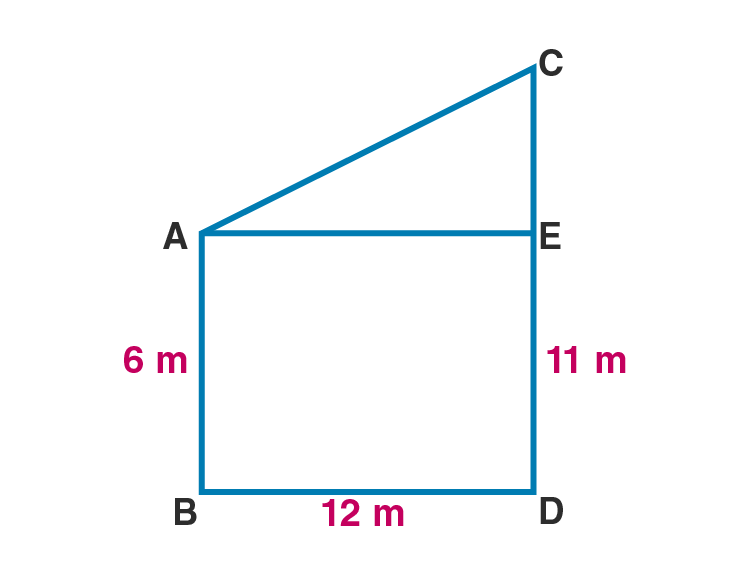Let AB and CD be the poles which are 12 m apart.

AB = 6 m

CD = 11 m

BD = 12 m

Draw AE BD

CE = 11-6 = 5 m

AE = 12 m

According to Pythagoras theorem,

AC2 = AE2+CE2

AC2 = 122+52

AC2 = 144+25

AC2 = 169

Taking square root on both sides

AC = 13

Hence the distance between their tops is 13 m.

5. In a right-angled triangle, if hypotenuse is 20 cm and the ratio of the other two sides is 4:3, find the sides.

Solution:

Given hypotenuse, h = 20 cm

Ratio of other two sides, a:b = 4:3

Let altitude of the triangle be 4x and base be 3x.

According to Pythagoras theorem,

h2 = b2+a2

202 = (3x)2+(4x)2

400 = 9x2+16x2

25x2 = 400

x2 = 400/25

x2 = 16

Taking square root on both sides

x = 4

so base, b = 3x = 3×4 = 12

altitude, a = 4x = 4×4 = 16

Hence the other sides are 12 cm and 16 cm.

6. If the sides of a triangle are in the ratio 3:4:5, prove that it is right-angled triangle.

Solution: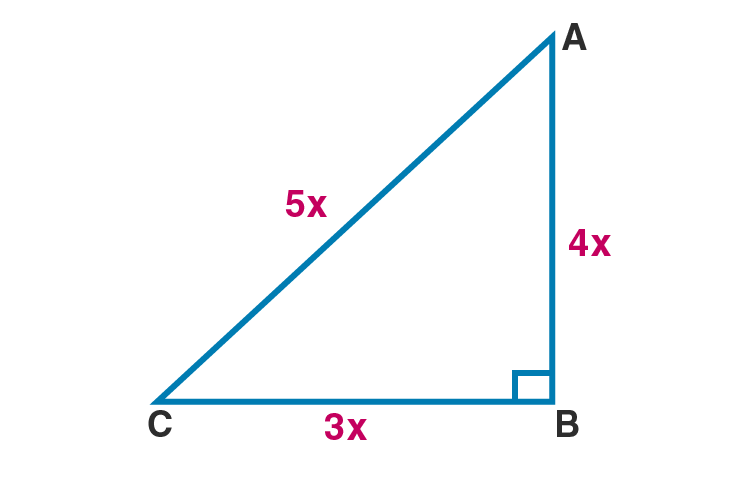Given the sides are in the ratio 3:4:5.

Let ABC be the given triangle.

Let the sides be 3x, 4x and hypotenuse be 5x.

According to Pythagoras theorem,

AC2 = BC2+AB2

BC2+AB2= (3x)2+(4x)2

= 9x2+16x2

= 25x2

AC2 = (5x)2 = 25x2

AC2 = BC2+AB2

Hence ABC is a right angled triangle.

7. For going to a city B from city A, there is route via city C such that AC ⊥ CB, AC = 2x km and CB=2(x+ 7) km. It is proposed to construct a 26 km highway which directly connects the two cities A and B. Find how much distance will be saved in reaching city B from city A after the construction of highway.

Solution: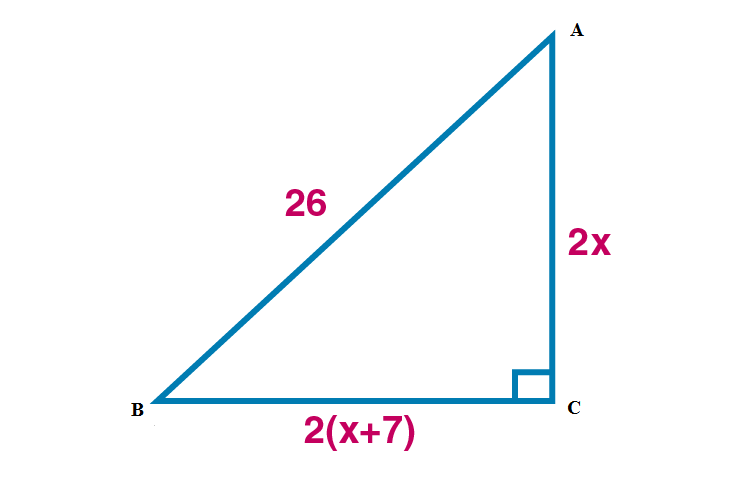Given AC = 2x km

CB = 2(x+7)km

AB = 26

Given AC CB.

According to Pythagoras theorem,

AB2 = CB2+AC2

262 = ( 2(x+7))2+(2x)2

676 = 4(x2+14x+49) + 4x2

4x2+56x+196+4x2 = 676

8x2+56x+196 = 676

8x2+56x +196-676 = 0

8x2+56x -480 = 0

x2+7x -60 = 0

(x-5)(x+12) = 0

(x-5) = 0 or (x+12) = 0

x = 5 or x = -12

Length cannot be negative. So x = 5

BC = 2(x+7) = 2(5+7) = 2×12 = 24 km

AC = 2x = 2×5 = 10 km

Total distance = AC + BC = 10+24 = 34 km

Distance saved = 34-26 = 8 km

Hence the distance saved is 8 km.

8. The hypotenuse of right triangle is 6m more than twice the shortest side. If the third side is 2m less than the hypotenuse, find the sides of the triangle.

Solution: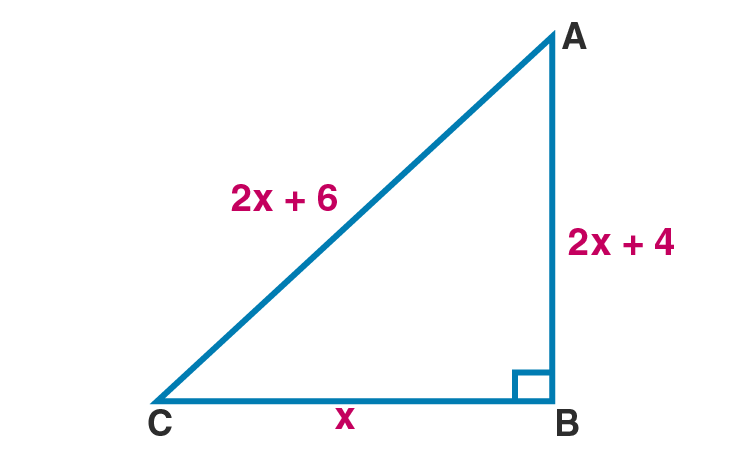Let the shortest side be x.

Then hypotenuse = 2x+6

Third side = 2x+6-2 = 2x+4

According to Pythagoras theorem,

AB2 = CB2+AC2

(2x+6)2 = x2+(2x+4)2

4x2+24x+36 = x2+4x2+16x+16

x2-8x-20 = 0

(x-10)(x+2) = 0

x-10 = 0 or x+2 = 0

x = 10 or x = -2

x cannot be negative.

So shortest side is 10 m.

Hypotenuse = 2x+6

= 2×10+6

= 20+6

= 26 m

Third side = 2x+4

= = 2×10+4

= 20+4

= 24 m

Hence the shortest side, hypotenuse and third side of the triangle are 10 m, 26 m and 24 m respectively.

9. ABC is an isosceles triangle right angled at C. Prove that AB² = 2AC².

Solution: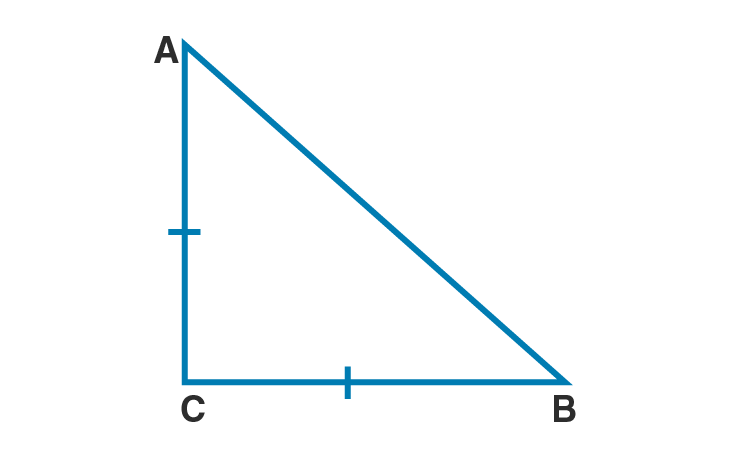Let ABC be the isosceles right angled triangle .

C = 90˚

AC = BC [isosceles triangle]

According to Pythagoras theorem,

AB2 = BC2+AC2

AB2 = AC2+AC2 [∵AC = BC]

AB2 = 2AC2

Hence proved.

10. In a triangle ABC, AD is perpendicular to BC. Prove that AB² + CD² = AC² + BD².

Solution: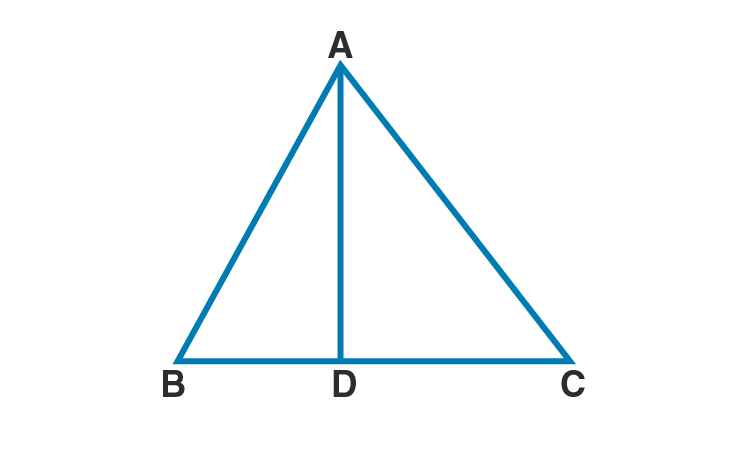Comparing (i) and (ii)

AB2– BD2 = AC2– CD2

AB2+ CD2 = AC2+ BD2

Hence proved.

11. In ∆PQR, PD ⊥ QR, such that D lies on QR. If PQ = a, PR = b, QD = c and DR = d,

prove that (a + b) (a – b) = (c + d) (c – d).

Solution: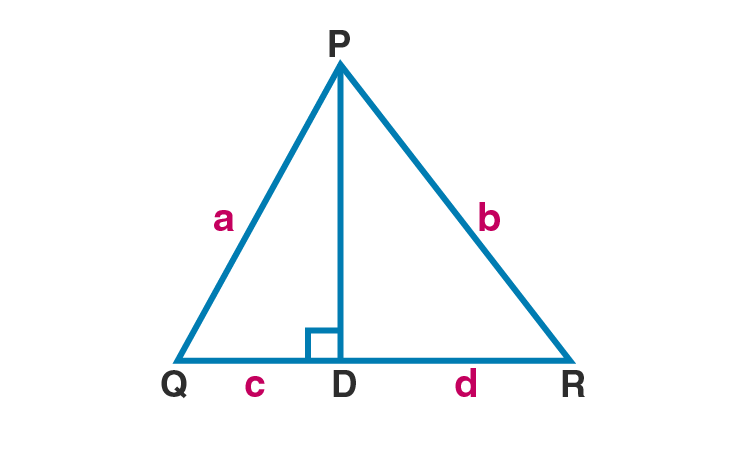Given PQ = a, PR = b, QD = c and DR = d.

PD QR.

So PDQ and PDR are right triangles.

In PDQ,

PQ2 = PD2+QD2 [Pythagoras theorem]

PD2 = PQ2– QD2

PD2 = a2– c2 …(i) [∵ PQ = a and QD = c]

In PDR,

PR2 = PD2+DR2 [Pythagoras theorem]

PD2 = PR2– DR2

PD2 = b2– d2 …(ii) [∵ PR = b and DR = d]

Comparing (i) and (ii)

a2– c2= b2– d2

a2– b2= c2– d2

(a+b)(a-b) = (c+d)(c-d)

Hence proved.

12. ABC is an isosceles triangle with AB = AC = 12 cm and BC = 8 cm. Find the altitude on BC and Hence, calculate its area.

Solution: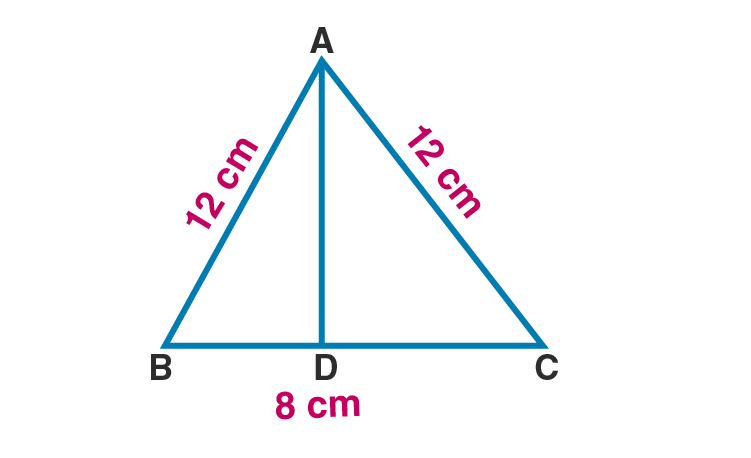Let AD be the altitude of ABC.

Given AB = AC = 12 cm

BC = 8 cm

The altitude to the base of an isosceles triangle bisects the base.

So BD = DC

BD = 8/2 = 4 cm

DC = 4 cm

AB2 = BD2 +AD2 [Pythagoras theorem]

Taking square root on both sides,

AD = √128 = √(2×64) = 8√2 cm

Area of ABC = ½ ×base ×height

= ½ ×8×8√2

= 4×8√2

= 32√2 cm2

Hence the area of triangle is 32√2 cm2.

13. Find the area and the perimeter of a square whose diagonal is 10 cm long.

Solution: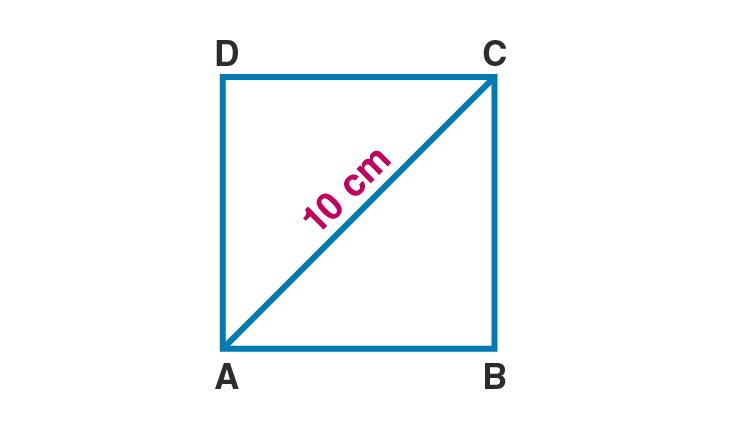Given length of the diagonal of the square is 10 cm.

AC = 10

Let AB = BC = x [Sides of square are equal in measure]

B = 90˚ [All angles of a square are 90˚]

ABC is a right triangle.

AC2 = AB2+BC2

102 = x2+x2

100 = 2x2

x2 = 50

x = √50 = √(25×2)

x = 5√2

So area of square = x2

= (5√2)2 = 50 cm2

Perimeter = 4x

= 4×5√2

= 20√2 cm

Hence area and perimeter of the square are 50 cm2 and 20√2 cm.

14. (a) In fig. (i) given below, ABCD is a quadrilateral in which AD = 13 cm, DC = 12 cm, BC = 3 cm, ∠ ABD = ∠BCD = 90°. Calculate the length of AB.
(b) In fig. (ii) given below, ABCD is a quadrilateral in which AB = AD, ∠A = 90° =∠C, BC = 8 cm and CD = 6 cm. Find AB and calculate the area of ∆ ABD.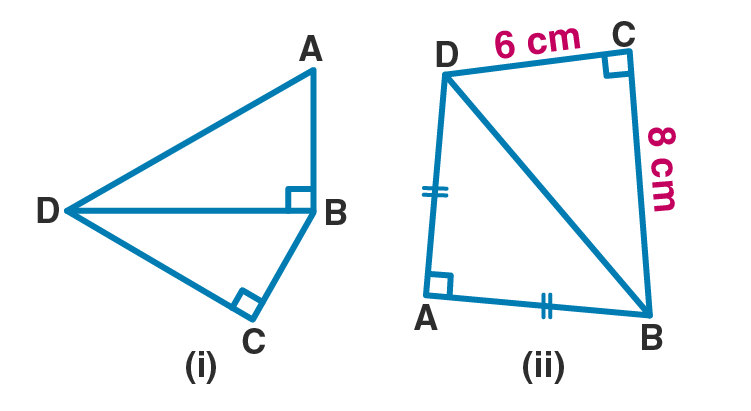Solution:

(i)Given AD = 13 cm, DC = 12 m

BC = 3 cm

ABD = BCD = 90˚

BCD is a right triangle.

BD2 = BC2+DC2 [Pythagoras theorem]

BD2 = 32+122

BD2 = 9+144

BD2 = 153

ABD is a right triangle.

132 = AB2+153

169 = AB2+153

AB2 = 169-153

AB2 = 16

Taking square root on both sides,

AB = 4 cm

Hence the length of AB is 4 cm.

(ii)Given AB = AD, A = 90° = C, BC = 8 cm and CD = 6 cm

BCD is a right triangle.

BD2 = BC2+DC2 [Pythagoras theorem]

BD2 = 82+62

BD2 = 64+36

BD2 = 100

Taking square root on both sides,

BD = 10 cm

ABD is a right triangle.

102 = 2AB2 [∵AB = AD]

100 = 2AB2

AB2 = 100/2

AB2 = 50

Taking square root on both sides,

AB = √50

AB = √(2×25)

AB = 5√2 cm

Hence the length of AB is 5√2 cm.

15. (a) In figure (i) given below, AB = 12 cm, AC = 13 cm, CE = 10 cm and DE = 6 cm. Calculate the length of BD.

(b) In figure (ii) given below, ∠PSR = 90°, PQ = 10 cm, QS = 6 cm and RQ = 9 cm. Calculate the length of PR.

(c) In figure (iii) given below, ∠ D = 90°, AB = 16 cm, BC = 12 cm and CA = 6 cm. Find CD.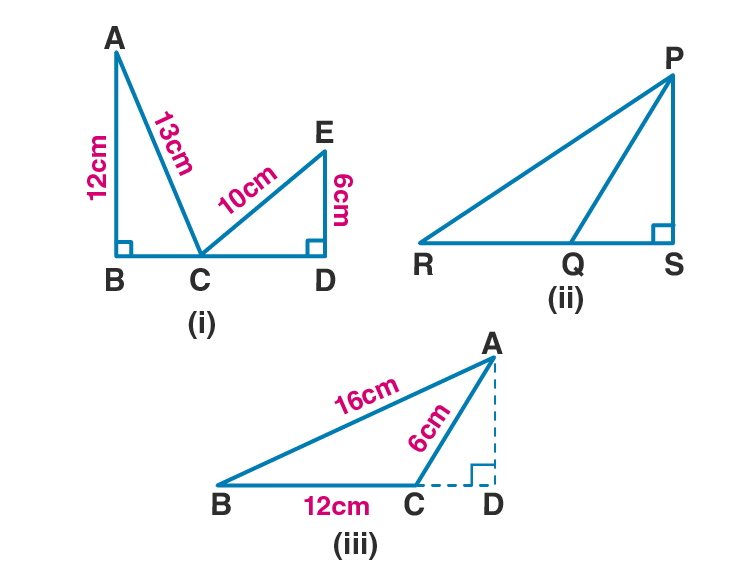Solution:

(a)Given AB = 12 cm, AC = 13 cm, CE = 10 cm and DE = 6 cm

ABC is a right triangle.

AC2 = AB2+BC2 [Pythagoras theorem]

132 = 122+BC2

BC2 = 132-122

BC2 = 169-144

BC2 = 25

Taking square root on both sides,

BC = 5 cm

CDE is a right triangle.

CE2 = CD2+DE2 [Pythagoras theorem]

102 = CD2+62

100 = CD2+36

CD2 = 100-36

CD2 = 64

Taking square root on both sides,

CD = 8 cm

BD = BC +CD

BD = 5+8

BD = 13 cm

Hence the length of BD is 13 cm.

(b) Given PSR = 90°, PQ = 10 cm, QS = 6 cm and RQ = 9 cm

PSQ is a right triangle.

PQ2 = PS2+QS2 [Pythagoras theorem]

102 = PS2+62

100 = PS2+36

PS2 = 100-36

PS2 = 64

Taking square root on both sides,

PS = 8 cm

PSR is a right triangle.

RS = RQ+QS

RS = 9+6

RS = 15 cm

PR2 = PS2+RS2 [Pythagoras theorem]

PR2 = 82+152

PR2 = 64+225

PR2 = 289

Taking square root on both sides,

PR = 17 cm

Hence the length of PR is 17 cm.

(c) D = 90°, AB = 16 cm, BC = 12 cm and CA = 6 cm

ABD is a right triangle.

62 = 112-24CD [from (i)]

36 = 112-24CD

24CD = 112-36

24CD = 76

CD = 76/24 = 19/6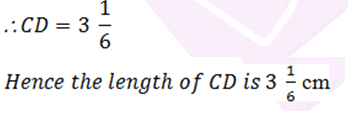16. (a) In figure (i) given below, BC = 5 cm,

∠B =90°, AB = 5AE, CD = 2AE and AC = ED. Calculate the lengths of EA, CD, AB and AC.

(b) In the figure (ii) given below, ABC is a right triangle right angled at C. If D is mid-point of BC, prove that AB2 = 4AD² – 3AC².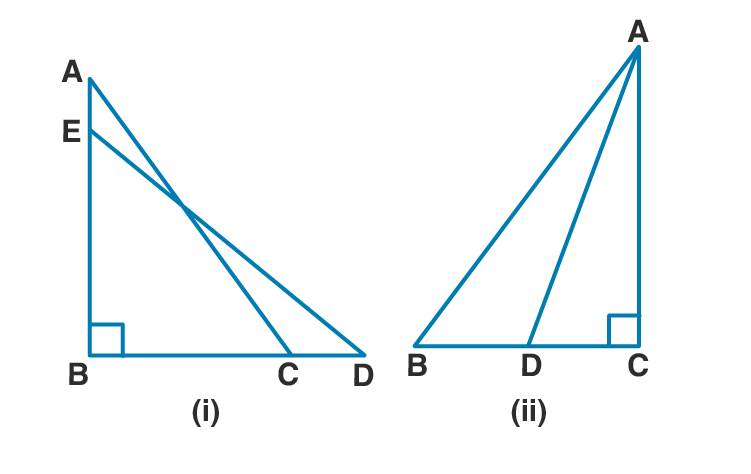Solution:

(a)Given BC = 5 cm,
B =90°, AB = 5AE,

CD = 2AE and AC = ED

ABC is a right triangle.

AC2 = AB2+BC2 …(i) [Pythagoras theorem]

BED is a right triangle.

ED2 = BE2+BD2 [Pythagoras theorem]

AC2 = BE2+BD2 …(ii) [∵AC = ED]

Comparing (i) and (ii)

AB2+BC2 = BE2+BD2

(5AE)2+52 = (4AE)2+(BC+CD)2 [∵BE = AB-AE = 5AE-AE = 4AE]

(5AE)2+25 = (4AE)2+(5+2AE)2 …(iii) [∵BC = 5, CD = 2AE]

Let AE = x. So (iii) becomes,

(5x)2+25 = (4x)2+(5+2x)2

25x2+25 = 16x2+25+20x+4x2

25x2 = 20x2+20x

5x2 = 20x

x = 20/5 = 4

AE = 4 cm

CD = 2AE = 2×4 = 8 cm

AB = 5AE

AB = 5×4 = 20 cm

ABC is a right triangle.

AC2 = AB2+BC2 [Pythagoras theorem]

AC2 = 202+52

AC2 = 400+25

AC2 = 425

Taking square root on both sides,

AC = √425 = √(25×17)

AC = 5√17 cm

Hence EA = 4 cm, CD = 8 cm, AB = 20 cm and AC = 5√17 cm.

(b)Given D is the midpoint of BC.

DC = ½ BC

ABC is a right triangle.

AB2 = AC2+BC2 …(i) [Pythagoras theorem]

AD2 = AC2+DC2 …(ii) [Pythagoras theorem]

AC2 = AD2– (½ BC)2 [∵DC = ½ BC]

Hence proved.

17. In ∆ ABC, AB = AC = x, BC = 10 cm and the area of ∆ ABC is 60 cm². Find x.

Solution: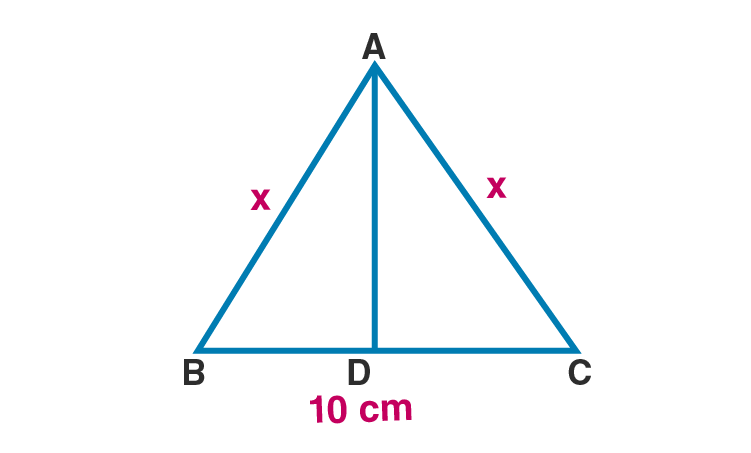Given AB = AC = x

So ABC is an isosceles triangle.

The altitude to the base of an isosceles triangle bisects the base.

BD = DC = 10/2 = 5 cm

Given area = 60 cm2

½ ×base ×height = ½ ×10×AD = 60

x2 = 122+52

x2 = 144+25

x2 = 169

Taking square root on both sides

x = 13 cm

Hence the value of x is 13 cm.

18. In a rhombus, If diagonals are 30 cm and 40 cm, find its perimeter.

Solution: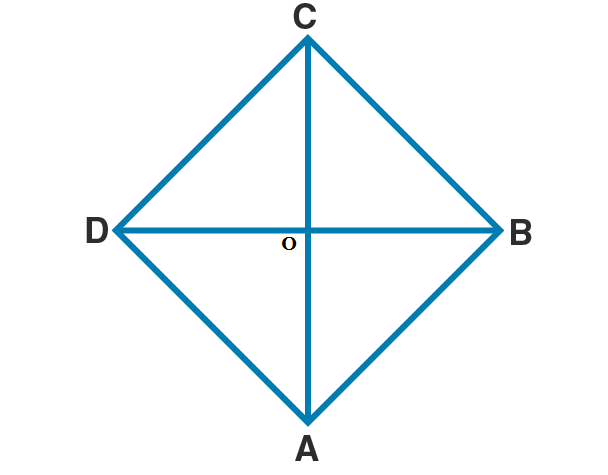Let ABCD be the rhombus.

Given AC = 30cm

BD = 40 cm

Diagonals of a rhombus are perpendicular bisectors of each other.

OB = ½ BD = ½ ×40 = 20 cm

OC = ½ AC = ½ ×30 = 15 cm

OCB is a right triangle.

BC2 = OC2+OB2 [Pythagoras theorem]

BC2 = 152+202

BC2 = 225+400

BC2 = 625

Taking square root on both sides

BC = 25 cm

So side of a rhombus, a = 25 cm.

Perimeter = 4a = 4×25 = 100 cm

Hence the perimeter of the rhombus is 100 cm.

19. (a) In figure (i) given below, AB || DC, BC = AD = 13 cm. AB = 22 cm and DC = 12cm. Calculate the height of the trapezium ABCD.

(b) In figure (ii) given below, AB || DC, ∠ A = 90°, DC = 7 cm, AB = 17 cm and AC = 25 cm. Calculate BC.

(c) In figure (iii) given below, ABCD is a square of side 7 cm. if

AE = FC = CG = HA = 3 cm,

(i) prove that EFGH is a rectangle.

(ii) find the area and perimeter of EFGH.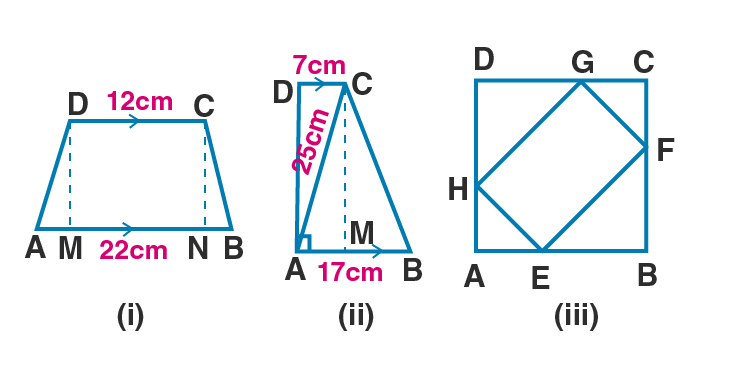Solution:

(i) Given AB || DC, BC = AD = 13 cm.

AB = 22 cm and DC = 12cm

Here DC = 12

MN = 12 cm

AM = BN

AB = AM+MN+BN

22 = AM+12+AM [∵AM = BN]

2AM = 22-12 = 10

AM = 10/2

AM = 5 cm

AMD is a right triangle.

132 = 52+DM2

DM2 = 132-52

DM2 = 169-25

DM2 = 144

Taking square root on both sides,

DM = 12 cm

Hence the height of the trapezium is 12 cm.

(b) Given AB || DC, A = 90°, DC = 7 cm,

AB = 17 cm and AC = 25 cm

Taking square root on both sides

CM = 24 cm [∵ABCD]

DC = 7 cm

AM = 7 cm

BM = AB-AM

BM = 17-7 = 10 cm

BMC is a right triangle.

BC2 = BM2+CM2

BC2 = 102+242

BC2 = 100+576

BC2 = 676

Taking square root on both sides

BC = 26 cm

Hence length of BC is 26 cm.

(c) (i)Proof: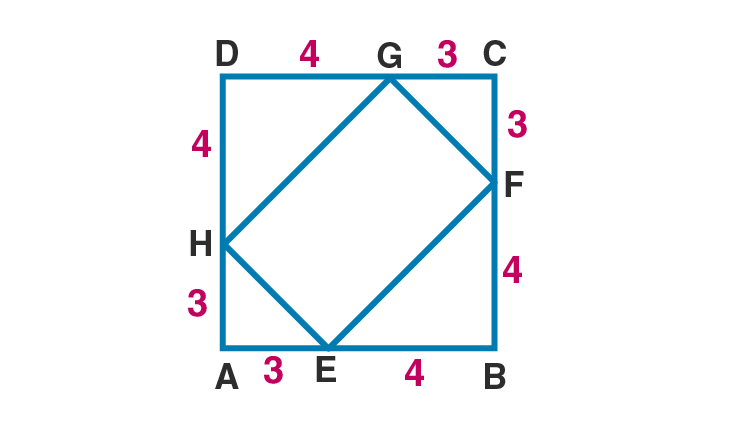Given ABCD is a square of side 7 cm.

So AB = BC = CD = AD = 7 cm

Also given AE = FC = CG = HA = 3 cm

BE = AB-AE = 7-3 = 4 cm

BF = BC-FC = 7-3 = 4 cm

GD = CD-CG = 7-3 = 4 cm

DH = AD-HA = 7-3 = 4 cm

A = 90˚ [Each angle of a square equals 90˚]

AHE is a right triangle.

HE2 = AE2+AH2 [Pythagoras theorem]

HE2 = 32+32

HE2 = 9+9 = 18

HE = √(9×2) = 3√2 cm

Similarly GF = 3√2 cm

EBF is a right triangle.

EF2 = BE2+BF2 [Pythagoras theorem]

EF2 = 42+42

EF2 = 16+16 = 32

Taking square root on both sides

EF = √(16×2) = 4√2 cm

Similarly HG = 4√2 cm

Now join EG

In EFG

EG2 = EF2+GF2

EG2 = (4√2)2+(3√2)2

EG2 = 32+18 = 50

EG = √50 = 5√2 cm …(i)

Join HF.

Also HF2 = EH2+HG2

= (3√2)2+(4√2)2

= 18+32 = 50

HF = √50 = 5√2 cm …(ii)

From (i) and (ii)

EG = HF

Diagonals of the quadrilateral are congruent. So EFGH is a rectangle.

Hence proved.

(ii)Area of rectangle EFGH = length × breadth

= HE ×EF

= 3√2×4√2

= 24 cm2

Perimeter of rectangle EFGH = 2(length+breadth)

= 2×(4√2+3√2)

= 2×7√2

= 14√2 cm

Hence area of the rectangle is 24 cm2 and perimeter is 14√2 cm.

20. AD is perpendicular to the side BC of an equilateral Δ ABC. Prove that 4AD² = 3AB².

Solution: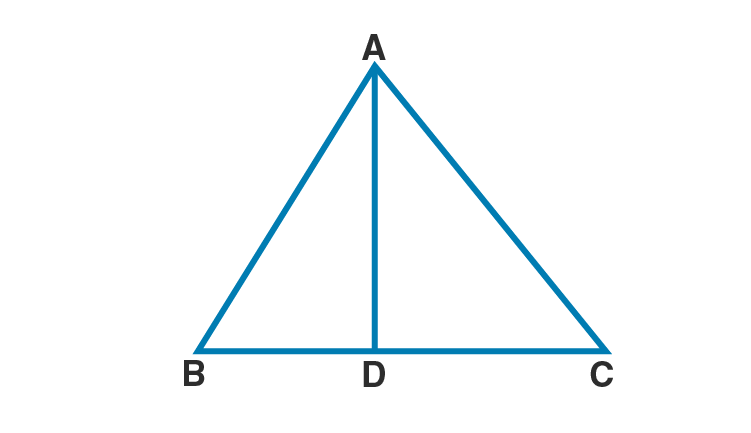D = 90˚

Proof:

Since ABC is an equilateral triangle,

AB = AC = BC

ABD is a right triangle.

According to Pythagoras theorem,

BD = ½ BC

AB2 = AD2+( ½ AB)2 [∵BC = AB]

Hence proved.

21. In figure (i) given below, D and E are mid-points of the sides BC and CA respectively of a ΔABC, right angled at C.

Prove that :

(ii)4BE2 = 4BC2+AC2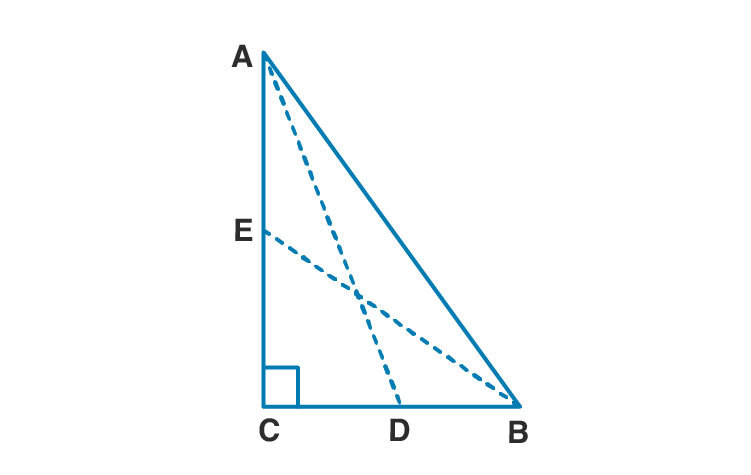Solution:

Proof:

(i)C = 90˚

So ACD is a right triangle.

Multiply both sides by 4, we get

4AD2 = 4AC2+4BD2 [∵D is the midpoint of BC, CD = BD = ½ BC]

4AD2 = 4AC2+BC2….(i) [∵BC = 2BD]

Hence proved.

(ii)BCE is a right triangle.

BE2 = BC2+CE2 [Pythagoras theorem]

Multply both sides by 4 , we get

4BE2 = 4BC2+4CE2

4BE2 = 4BC2+(2CE)2

4BE2 = 4BC2+AC2 ….(ii) [∵E is the midpoint of AC, AE = CE = ½ AC]

Hence proved.

4(AD2+BE2 ) = 5(AB2) [∵ABC is a right triangle, AB2 = AC2+BC2]

Hence proved.

22. If AD, BE and CF are medians of ABC, prove that 3(AB² + BC² + CA²) = 4(AD² + BE² + CF²).

Solution: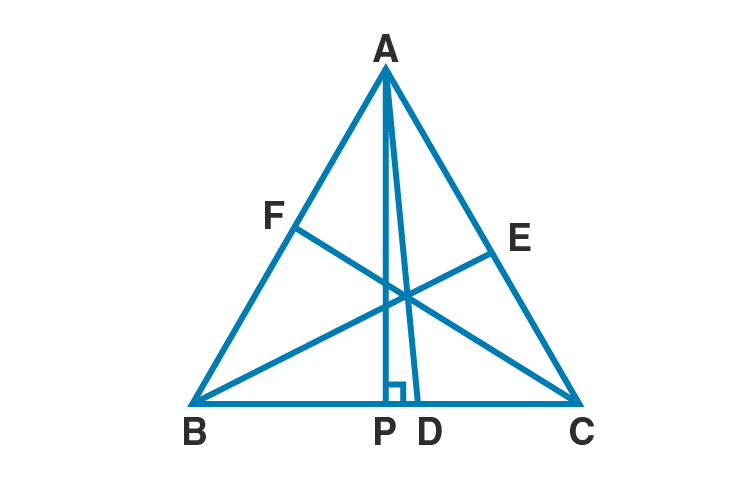Construction:

Draw APBC

Proof:

APB is a right triangle.

AB2 = AP2+BP2 [Pythagoras theorem]

AB2 = AP2+(BD-PD)2

AB2 = AP2+BD2+PD2-2BD×PD

AB2 = (AP2+PD2)+BD2-2BD×PD

AB2 = AD2+ (½ BC)2-2×( ½ BC)×PD [∵AP2+PD2 = AD2 and BD = ½ BC]

AB2 = AD2+ ¼ BC2– BC×PD ….(i)

APC is a right triangle.

AC2 = AP2+PC2 [Pythagoras theorem]

AC2 = AP2+(PD2+DC2)

AC2 = AP2+PD2+DC2+2×PD×DC

AC2 = (AP2+PD2)+ (½ BC)2+2×PD×( ½ BC) [DC = ½ BC]

AC2 = (AD)2+ ¼ BC2+PD× BC …(ii) [In APD, AP2+PD2 = AD2]

Adding (i) and (ii), we get

AB2+AC2 = 2AD2+ ½ BC2 …..(iii)

Draw perpendicular from B and C to AC and AB respectively.

Similarly we get,

BC2+CA2 = 2CF2+ ½ AB2 ….(iv)

AB2+BC2 = 2BE2+ ½ AC2 ….(v)

Adding (iii), (iv) and (v), we get

2(AB2+BC2+CA2) = 2(AB2+BC2+CA2) – ½ (AB2+BC2+CA2)

Hence proved.

23.(a) In fig. (i) given below, the diagonals AC and BD of a quadrilateral ABCD intersect at O, at right angles. Prove that AB² + CD² = AD² + BC².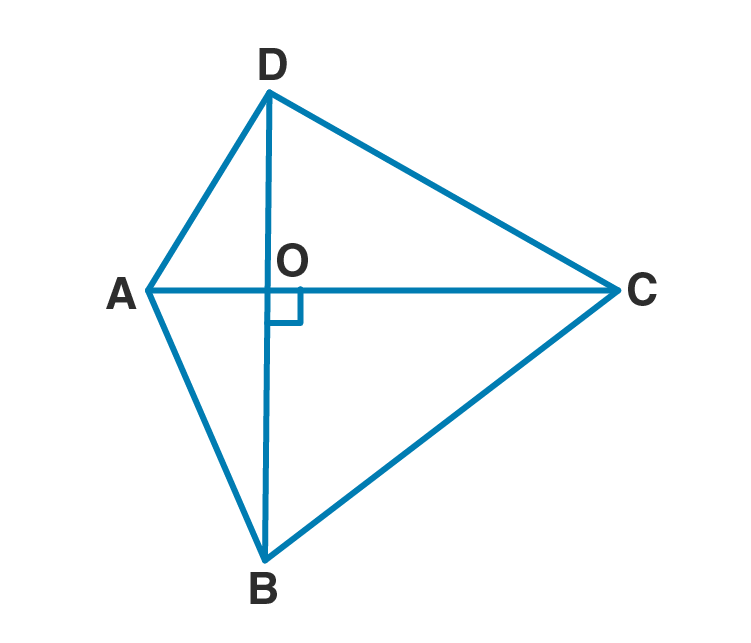Solution:

Given diagonals of quadrilateral ABCD, AC and BD intersect at O at right angles.

Proof:

AOB is a right triangle.

AB2 = OB2+OA2 …(i) [Pythagoras theorem]

COD is a right triangle.

CD2 = OC2+OD2 …(ii) [Pythagoras theorem]

Adding (i) and (ii), we get

AB2+ CD2 = OB2+OA2+ OC2+OD2

AB2+ CD2 = (OA2+OD2)+ (OC2+OB2) …(iii)

AOD is a right triangle.

AD2 = OA2+OD2 …(iv) [Pythagoras theorem]

BOC is a right triangle.

BC2 = OC2+OB2 …(v) [Pythagoras theorem]

Substitute (iv) and (v) in (iii), we get

Hence proved.

24. In a quadrilateral ABCD, B = 90° = D. Prove that 2 AC² – BC2 = AB² + AD² + DC².

Solution: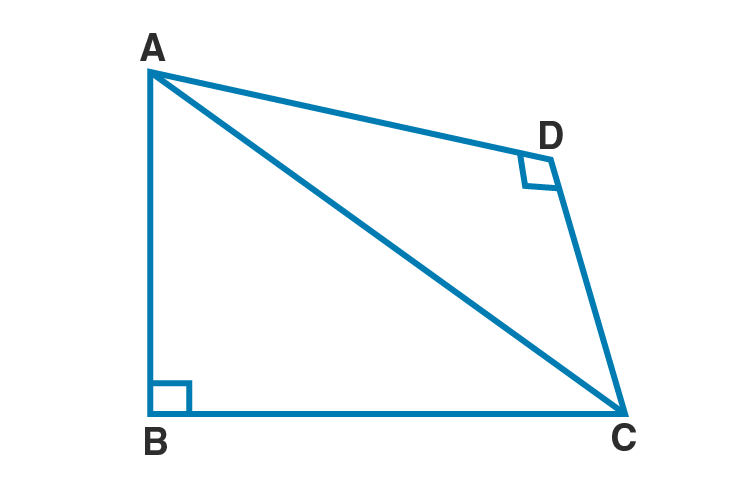Given B = D = 90˚

So ABC and ADC are right triangles.

In ABC,

AC2 = AB2+BC2 …(i) [Pythagoras theorem]

AC2 = AD2+DC2 …(ii) [Pythagoras theorem]

Hence proved.

25. In a ∆ ABC, A = 90°, CA = AB and D is a point on AB produced. Prove that : DC² – BD² = 2AB×AD.

Solution: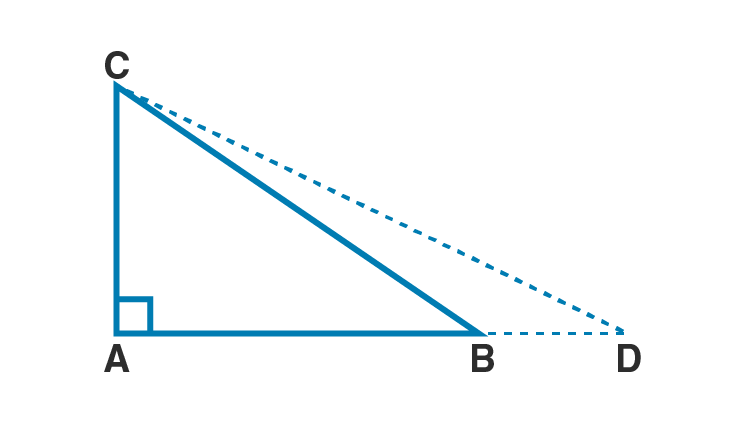Given A = 90˚

CA = AB

Proof:

In ACD,

DC2 = CA2+(AB+BD)2

DC2 = CA2+AB2+BD2+2AB×BD

DC2 -BD2 = CA2+AB2+2AB×BD

DC2 -BD2 = AB2+AB2+2AB×BD [∵CA = AB]

DC2 -BD2 = 2AB2+2AB×BD

DC2 -BD2 = 2AB(AB+BD)

Hence proved.

26. In an isosceles triangle ABC, AB = AC and D is a point on BC produced.

Solution: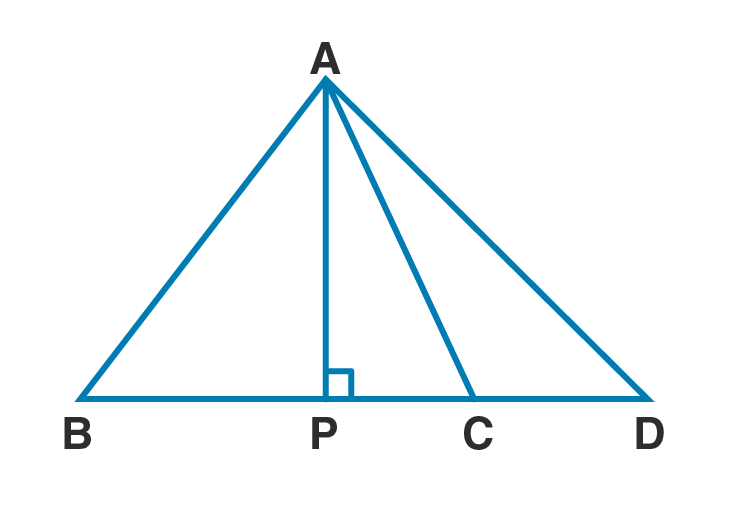Given ABC is an isosceles triangle.

AB = AC

Construction: Draw AP BC

Proof:

APD is a right triangle.

AD2 = AP2+(PC+CD)2 [PD = PC+CD]

APC is a right triangle.

AC2 = AP2+PC2 …(ii) [Pythagoras theorem]

Substitute (ii) in (i)

Since ABC is an isosceles triangle,

PC = ½ BC [The altitude to the base of an isosceles triangle bisects the base]

AD2 = AC2 +CD2+2× ½ BC ×CD

AD2 = AC2 +CD×BD [CD+BC = BD]

Hence proved.

Chapter test

1. a) In fig. (i) given below, AD ⊥ BC, AB = 25 cm, AC = 17 cm and AD = 15 cm. Find the length of BC.

(b) In figure (ii) given below, BAC = 90°, ADC = 90°, AD = 6 cm, CD = 8 cm and BC = 26 cm.

Find :(i) AC

(ii) AB

(iii) area of the shaded region.

(c) In figure (iii) given below, triangle ABC is right angled at B. Given that AB = 9 cm, AC = 15 cm and D, E are mid-points of the sides AB and AC respectively, calculate

(i) the length of BC

(ii) the area of ∆ ADE.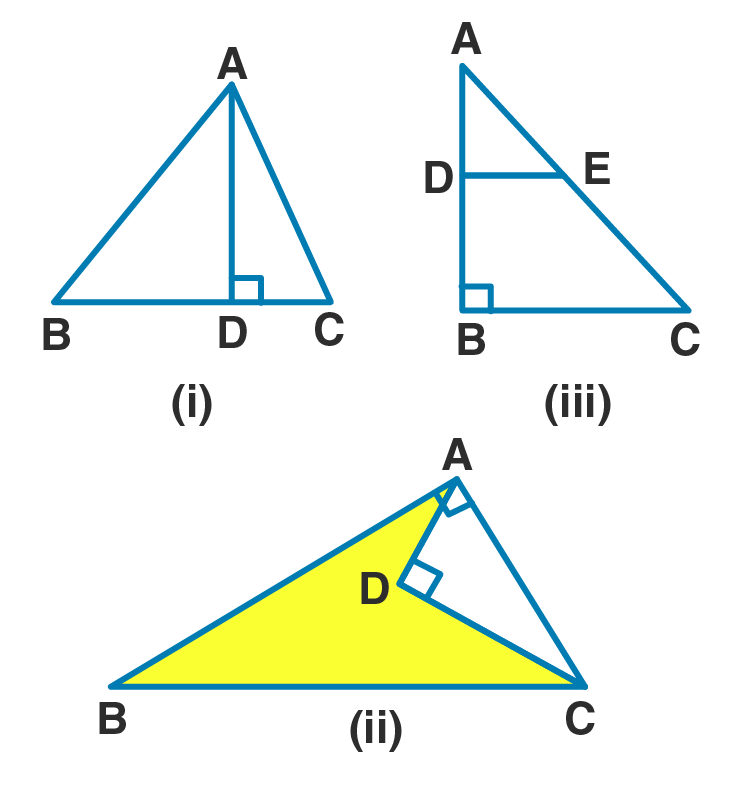Solution:

(a) Given AD ⊥ BC, AB = 25 cm, AC = 17 cm and AD = 15 cm

172 = 152+DC2

289 = 225+DC2

DC2 = 289-225

DC2 = 64

Taking square root on both sides,

DC = 8 cm

252 = 152+BD2

625 = 225+ BD2

BD2 = 625-225 = 400

Taking square root on both sides,

BD = 20 cm

BC = BD+DC

= 20+8

= 28 cm

Hence the length of BC is 28 cm.

(b) Given BAC = 90°, ADC = 90°

AD = 6 cm, CD = 8 cm and BC = 26 cm.

(i) ADC is a right triangle.

AC2 = 62+82

AC2 = 36+64

AC2 = 100

Taking square root on both sides,

AC = 10 cm

Hence length of AC is 10 cm.

(ii) ABC is a right triangle.

BC2 = AC2+AB2 [Pythagoras theorem]

262 = 102+AB2

AB2 = 262-102

AB2 = 676-100

AB2 = 576

Taking square root on both sides,

AB = 24 cm

Hence length of AB is 24 cm.

(iii)Area of ABC = ½ ×AB×AC

= ½ ×24×10

= 120 cm2

= ½ ×6×8

= 24 cm2

= 120-24

= 96 cm2

Hence the area of shaded region is 96 cm2.

(c) Given B = 90˚.

AB = 9 cm, AC = 15 cm .

D, E are mid-points of the sides AB and AC respectively.

(i)ABC is a right triangle.

AC2 = AB2+BC2 [Pythagoras theorem]

152 = 92+BC2

225 = 81+BC2

BC2 = 225-81

BC2 = 144

Taking square root on both sides,

BC = 12 cm

Hence the length of BC is 12 cm.

(ii) AD = ½ AB [D is the midpoint of AB]

AD = ½ ×9 = 9/2

AE = ½ AC [E is the midpoint of AC]

AE = ½ ×15 = 15/2

(15/2)2 = (9/2)2+DE2

DE2 = (15/2)2 – (9/2)2

DE2 = 225/4 -81/4

DE2 = 144/4

Taking square root on both sides,

DE = 12/2 = 6 cm.

= ½ ×6×9/2

= 13.5 cm2

Hence the area of the ADE is 13.5 cm2.

2. If in ∆ ABC, AB > AC and AD BC, prove that AB² – AC² = BD² – CD²

Solution: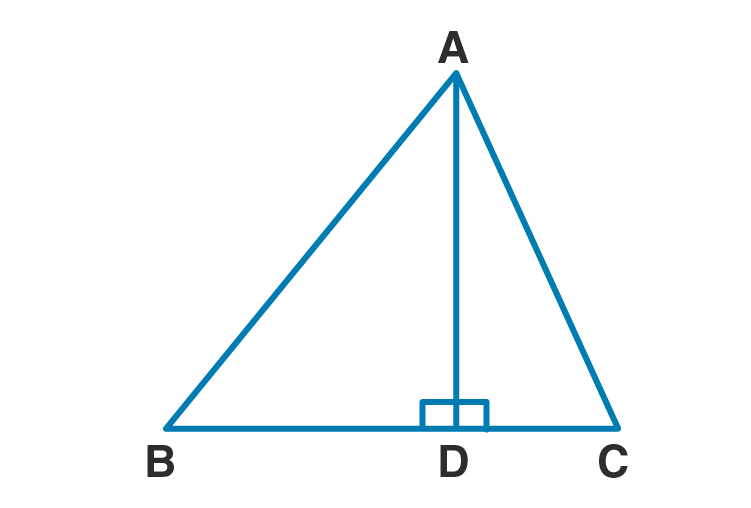Proof:

Equating (i) and (ii)

AB2-BD2 = AC2-CD2

AB2-AC2 = BD2– CD2

Hence proved.

3. In a right angled triangle ABC, right angled at C, P and Q are the points on the sides CA and CB respectively which divide these sides in the ratio 2:1.

Prove that

(i) 9AQ² = 9AC² + 4BC²

(ii) 9BP² = 9BC² + 4AC²

(iii) 9(AQ² + BP²) = 13AB².

Solution: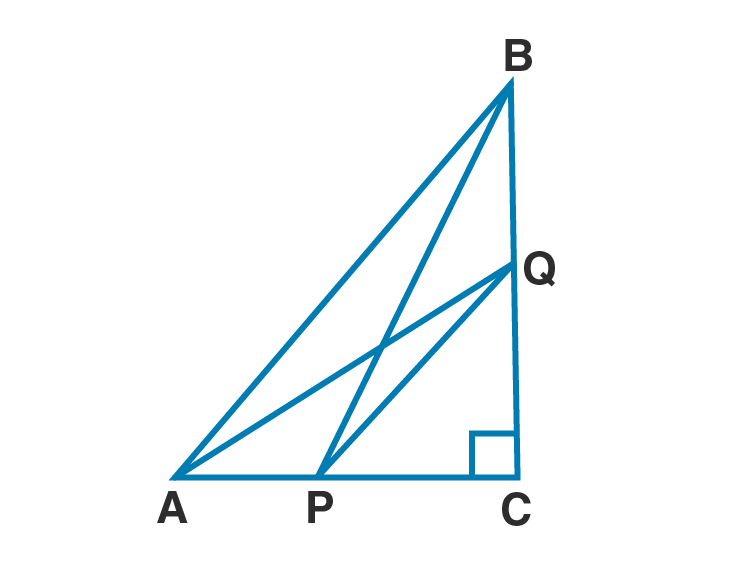Construction:

Join AQ and BP.

Given C = 90˚

Proof:

(i) In ACQ,

AQ2 = AC2+CQ2 [Pythagoras theorem]

Multiplying both sides by 9, we get

9AQ2 = 9AC2+9CQ2

9AQ2 = 9AC2+(3CQ)2 …(i)

Given BQ: CQ = 1:2

CQ/BC = CQ/(BQ+CQ)

CQ/BC = 2/3

3CQ = 2BC ….(ii)

Substitute (ii) in (i)

9AQ2 = 9AC2+(2BC)2

9AQ2 = 9AC2+4BC2 …(iii)

Hence proved.

(ii) ) In BPC,

BP2 = BC2+CP2 [Pythagoras theorem]

Multiplying both sides by 9, we get

9BP2 = 9BC2+9CP2

9BP2 = 9BC2+(3CP)2 …(iv)

Given AP: PC = 1:2

CP/AC = CP/AP+PC

CP/AC = 2/3

3CP = 2AC ….(v)

Substitute (v) in (iv)

9BP2 = 9BC2+(2AC)2

9BP2 = 9BC2+4AC2 ..(vi)

Hence proved.

(iii)Adding (iii) and (vi), we get

9AQ2+9BP2 = 9AC2+4BC2+9BC2+4AC2

9(AQ2+BP)2 = 13AC2+13BC2

9(AQ2+BP)2 = 13(AC2+BC2)…(vii)

In ABC,

AB2 = AC2+BC2 …..(viii)

Substitute (viii) in (viii), we get

9(AQ2+BP)2 = 13AB2

Hence proved.

4. In the given figure, ∆PQR is right angled at Q and points S and T trisect side QR. Prove that 8PT² = 3PR² + 5PS².

Solution: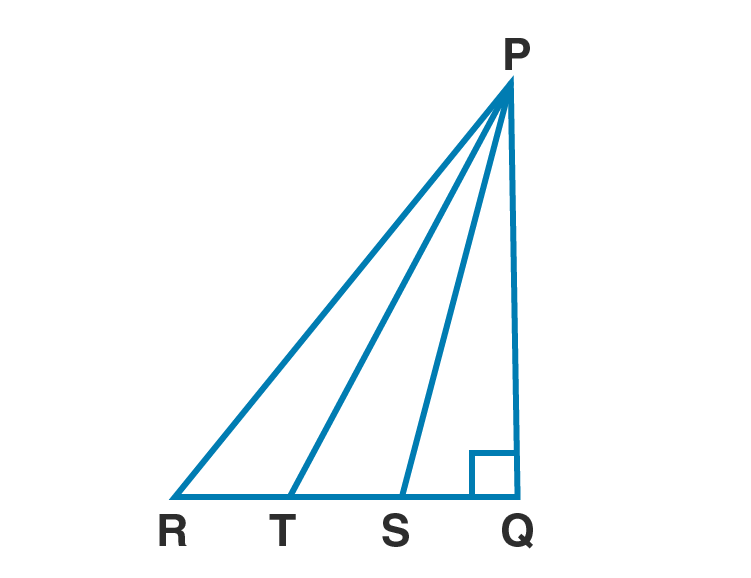Given Q = 90˚

S and T are points on RQ such that these points trisect it.

So RT = TS = SQ

To prove : 8PT² = 3PR² + 5PS².

Proof:

Let RT = TS = SQ = x

In PRQ,

PR2 = RQ2+PQ2 [Pythagoras theorem]

PR2 = (3x)2+PQ2

PR2 = 9x2+PQ2

Multiply above equation by 3

3PR2 = 27x2+3PQ2 ….(i)

Similarly in PTS,

PT2 = TQ2+PQ2 [Pythagoras theorem]

PT2 = (2x)2+PQ2

PT2 = 4x2+PQ2

Multiply above equation by 8

8PT2 = 32x2+8PQ2 ….(ii)

Similarly in PSQ,

PS2 = SQ2+PQ2 [Pythagoras theorem]

PS2 = x2+PQ2

Multiply above equation by 5

5PS2 = 5x2+5PQ2 …(iii)

Add (i) and (iii), we get

3PR2 +5PS2 = 27x2+3PQ2+5x2+5PQ2

3PR2 +5PS2 = 32x2+8PQ2

3PR2 +5PS2 = 8PT2 [From (ii)]

8PT2 = 3PR2 +5PS2

Hence proved.

5. In a quadrilateral ABCD, B = 90°. If AD² = AB² + BC² + CD², prove that ACD = 90°.

Solution: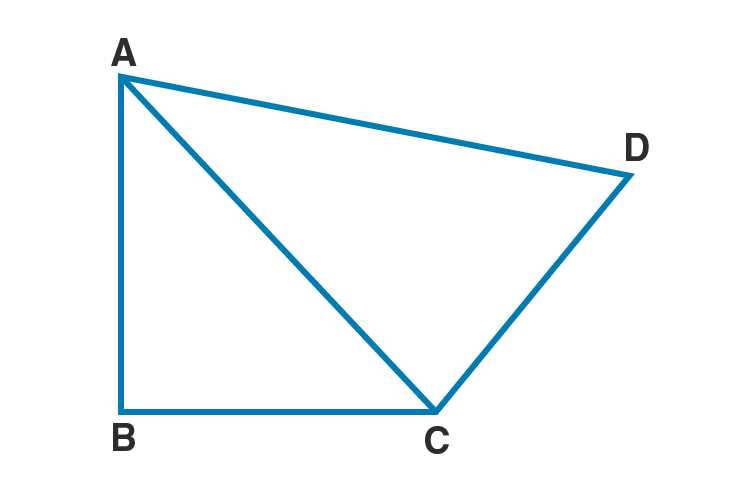Given : B = 90˚ in quadrilateral ABCD

AD² = AB² + BC² + CD²

To prove: ACD = 90˚

Proof:

In ABC,

AC2 = AB2+BC2 ….(i) [Pythagoras theorem]

Given AD² = AB² + BC² + CD²

In ACD, ACD = 90˚ [Converse of Pythagoras theorem]

Hence proved.

6. In the given figure, find the length of AD in terms of b and c.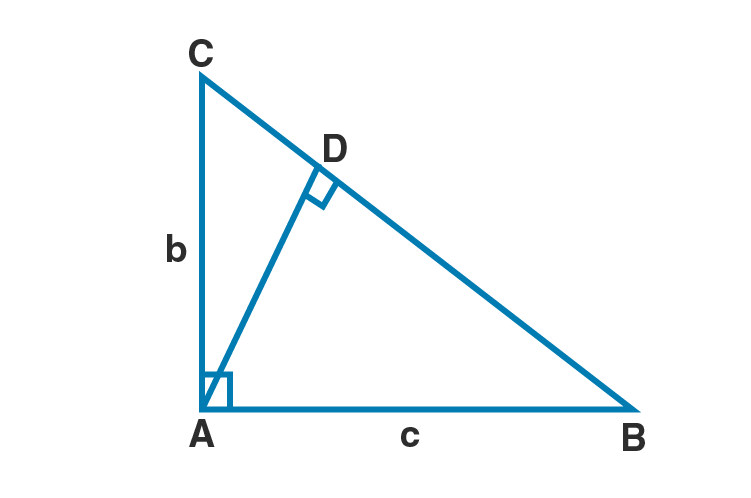Solution:

Given : A = 90˚

AB = c

AC = b

In ABC,

BC2 = AC2+AB2 [Pythagoras theorem]

BC2 = b2+c2

BC = √( b2+c2) …(i)

Area of ABC = ½ ×AB×AC

= ½ ×bc …(ii)

Also, Area of ABC = ½ ×BC×AD

= ½ ×√( b2+c2) ×AD …(iii)

Equating (ii) and (iii)

½ ×bc = ½ ×√( b2+c2) ×AD

Hence AD is bc /(√( b2+c2).

7. ABCD is a square, F is mid-point of AB and BE is one-third of BC. If area of ∆FBE is 108 cm², find the length of AC.

Solution: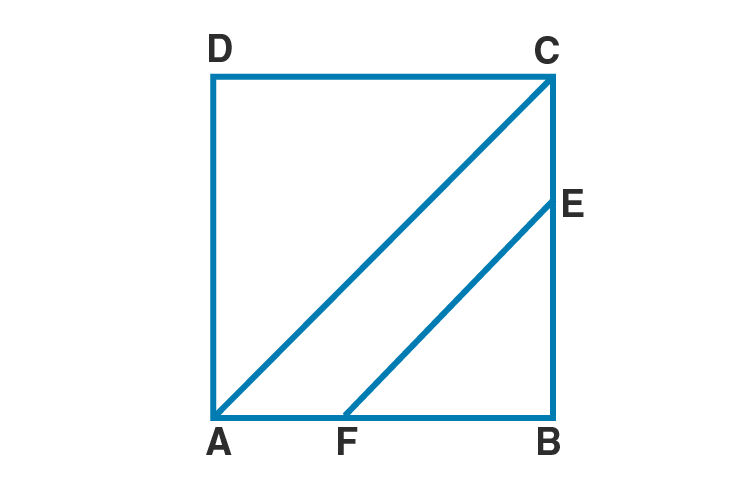Let x be each side of the square ABCD.

FB = ½ AB [∵ F is the midpoint of AB]

FB = ½ x …(i)

BE = (1/3) BC

BE = (1/3) x …(ii)

AC = √2 ×side [Diagonal of a square]

AC = √2x

Area of FBE = ½ FB×BE

108 = ½ × ½ x ×(1/3)x [given area of FBE = 108 cm2]

108 = (1/12)x2

x2 = 108×12

x2 = 1296

Taking square root on both sides.

x = 36

AC = √2×36 = 36√2

Hence length of AC is 36√2 cm.

8. In a triangle ABC, AB = AC and D is a point on side AC such that BC² = AC × CD, Prove that BD = BC.

Solution: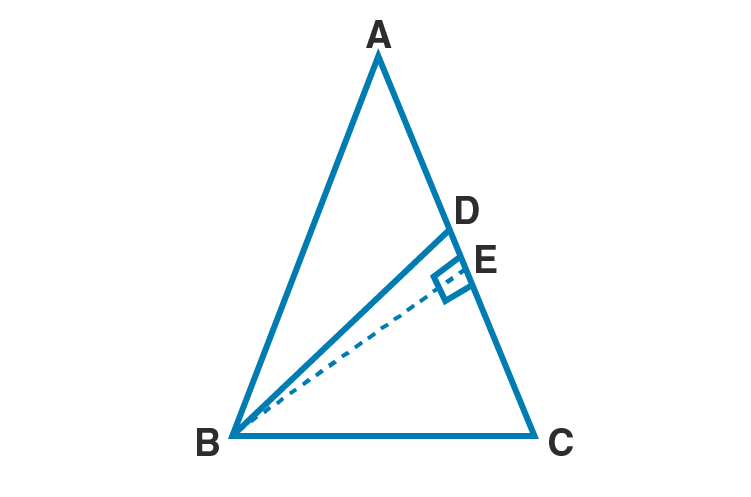Given : In ABC, AB = AC

D is a point on side AC such that BC² = AC × CD

To prove : BD = BC

Construction: Draw BEAC

Proof:

In BCE ,

BC2 = BE2+EC2 [Pythagoras theorem]

BC2 = BE2+(AC-AE)2

BC2 = BE2+AC2+AE2-2 AC×AE

BC2 = BE2+AE2+AC2-2 AC×AE …(i)

In ABC,

AB2 = BE2+AE 2 ..(ii)

Substitute (ii) in (i)

BC2 = AB2+AC2-2 AC×AE

BC2 = AC2+AC2-2 AC×AE [∵AB = AC]

BC2 = 2AC2-2 AC×AE

BC2 = 2AC(AC-AE)

BC2 = 2AC×EC

Given BC² = AC × CD

2AC×EC = AC × CD

2EC = CD ..(ii)

E is the midpoint of CD.

EC = DE …(iii)

In BED and BEC,

EC = DE [From (iii)]

BE = BE [common side]

BED = BEC

BED BEC [By SAS congruency rule]

BD = BD [c.p.c.t]

Hence proved.